# 数据结构与算法 复习

## REVISION

Posted by R1NG on January 5, 2022 Viewed Times

# COMP26120 REVISION

## 1. 算法设计与复杂度分析

### 1.1 算法时间复杂度分析的基本方法和基本定义

#### 1. 算法时间复杂度的粗略分析: 以冒泡排序为例

1
2
3
4
5
6
7
8
9
10
for(i=0; i<N-1; i++) {
for(j=0; j<N-1; j++) {
if (a[j] > a[j+1]) {
t = a[j];
a[j] = a[j+1];
a[j+1] = t;
}
}
}



#### 2. 算法的渐进性能: 以线性查找和二分查找为例

1
2
3
4
5
6
7
8
9
10
11
j=1;

while (j <= A.length && A[j] != q) {
j++;
}

if (j < length(A)) {
return j;
} else {
return null;
}


1
2
3
4
5
6
7
8
9
10
11
12
13
l = 1;
r = A.length;
while (l <= r) {
j = l + (r - l)/2;
if (A[j] == target) {
return j;
} else if (A[j] > target) {
r = j - 1;
} else {
l = j + 1;
}
}
return null;


1. 任何内存地址的访问 消耗的时间和其他资源 相同.
2. 不考虑指令并行执行的情况.
3. 除了对函数的调用以外, 所有的指令执行时间 相同.
4. 除非特殊指定, 否则规定字长 (word size) 为某个常数.

#### 3. 研究算法的时间复杂度: 以插入排序为例

1
2
3
4
5
6
7
8
9
10
11
InsertionSort(A, n) {
for (i=2; i<n; i++) {
key = A[i];
j = i-1;
while (j>0 && A[j] > key) {
A[j+1] = A[j];
j -= 1;
}
A[j+1] = key;
}
}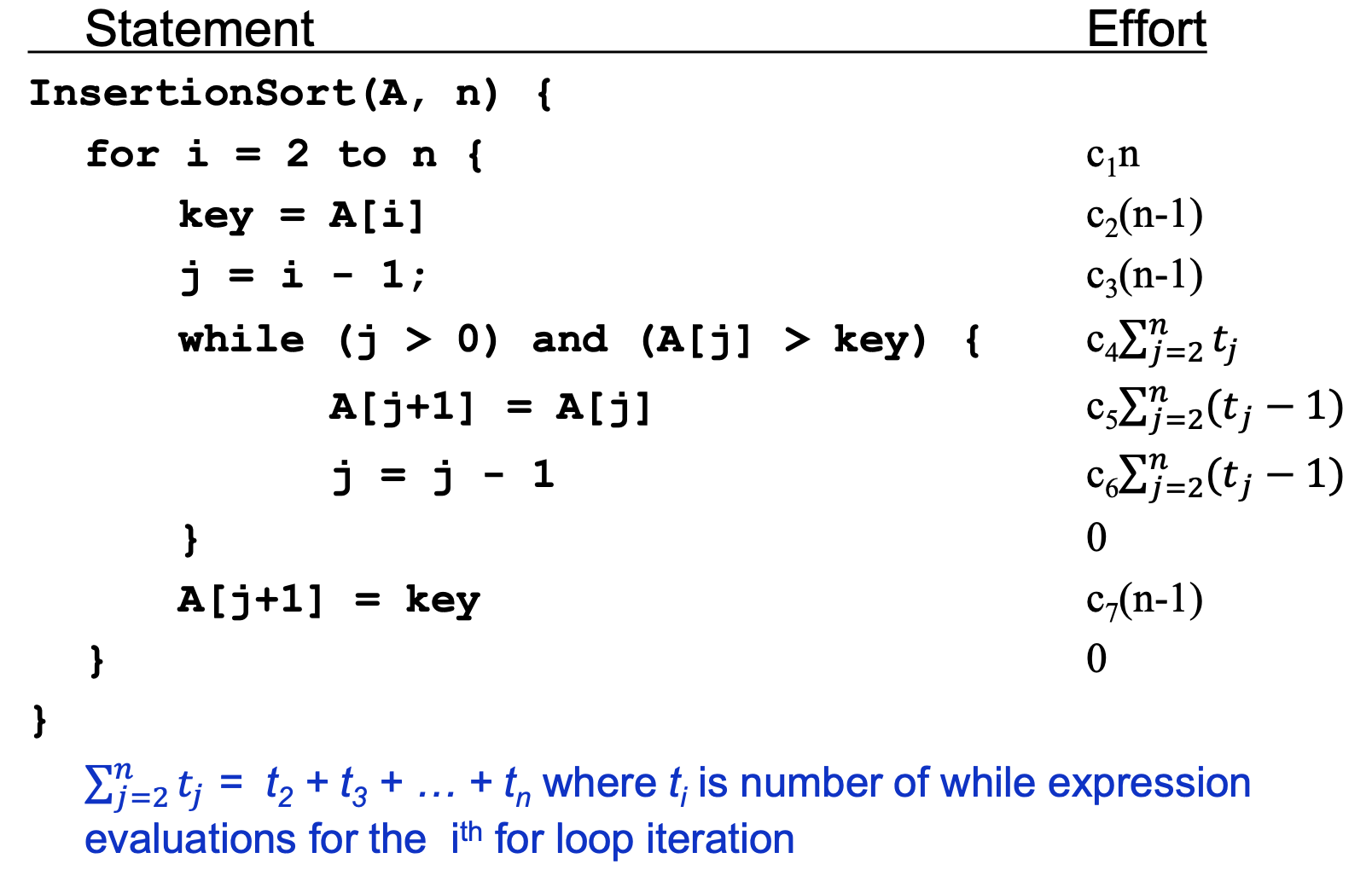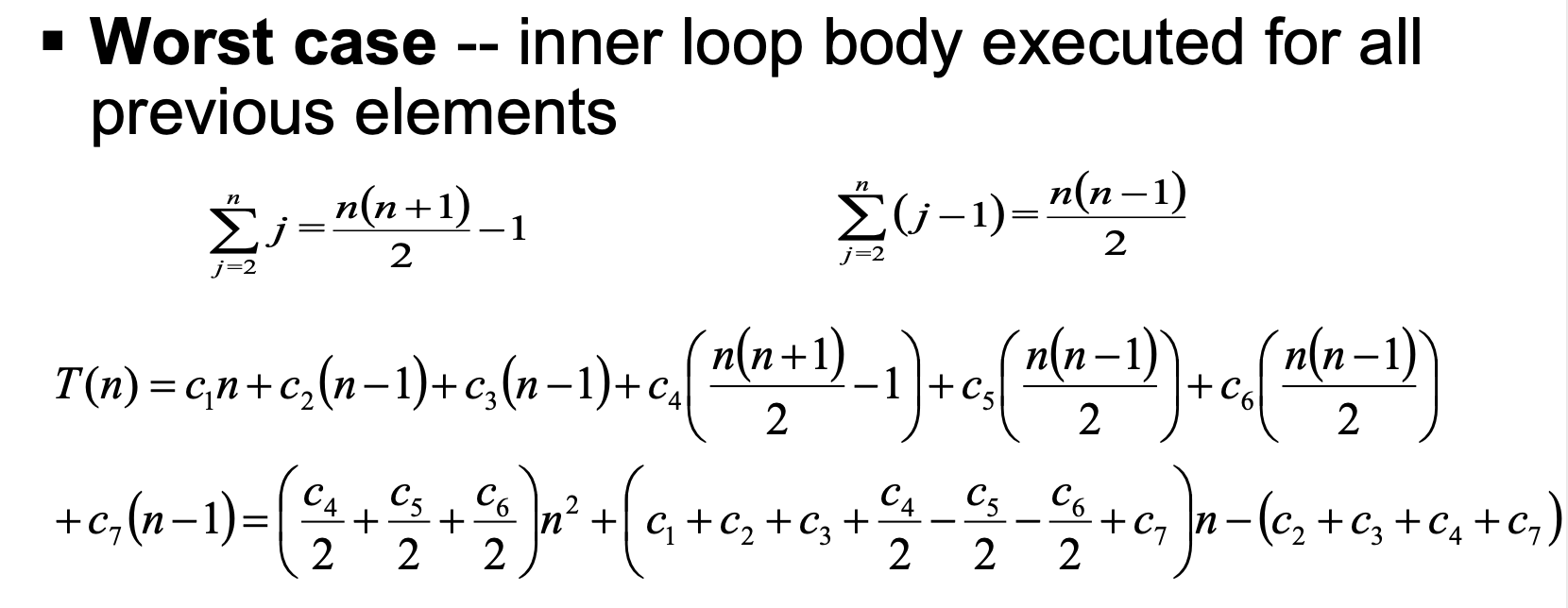#### 4. 对基本记号和概念的定义

$O(g(n)) = \{f(n) ~:~ \exists c > 0, n_0 > 0, ~\text{s.t.} ~ \forall n \geqslant n_0; 0 \leqslant f(n) \leqslant c \cdot g(n)\}.$

$\Omega(g(n)) = \{f(n) ~:~ \exists c > 0, n_0 > 0, ~\text{s.t.} ~ \forall n \geqslant n_0; 0 \leqslant c \cdot g(n) \leqslant f(n)\}.$

$\Theta(g(n)) = \{f(n) ~:~ \exists c_1, c_2 > 0, n_0 > 0, ~\text{s.t.} ~ \forall n \geqslant n_0; 0 \leqslant c_1 \cdot g(n) \leqslant f(n) \leqslant c_2 \cdot g(n)\}.$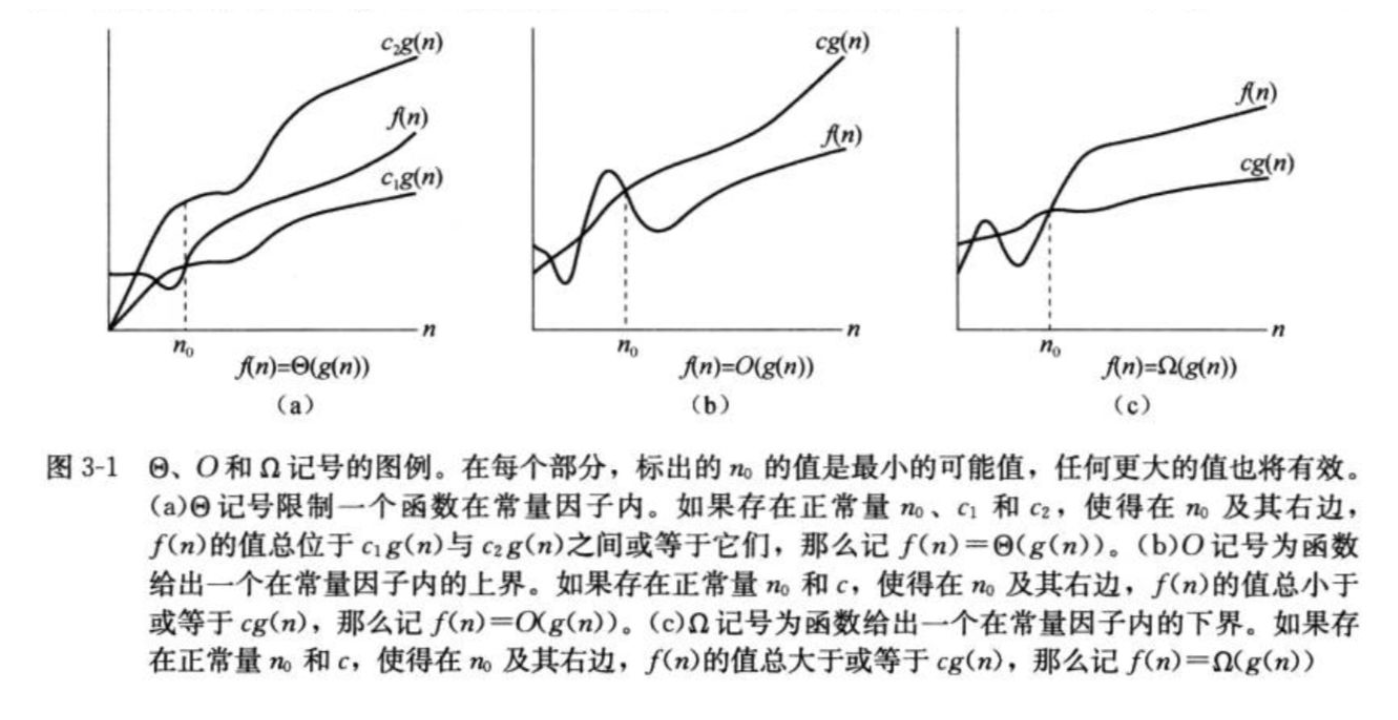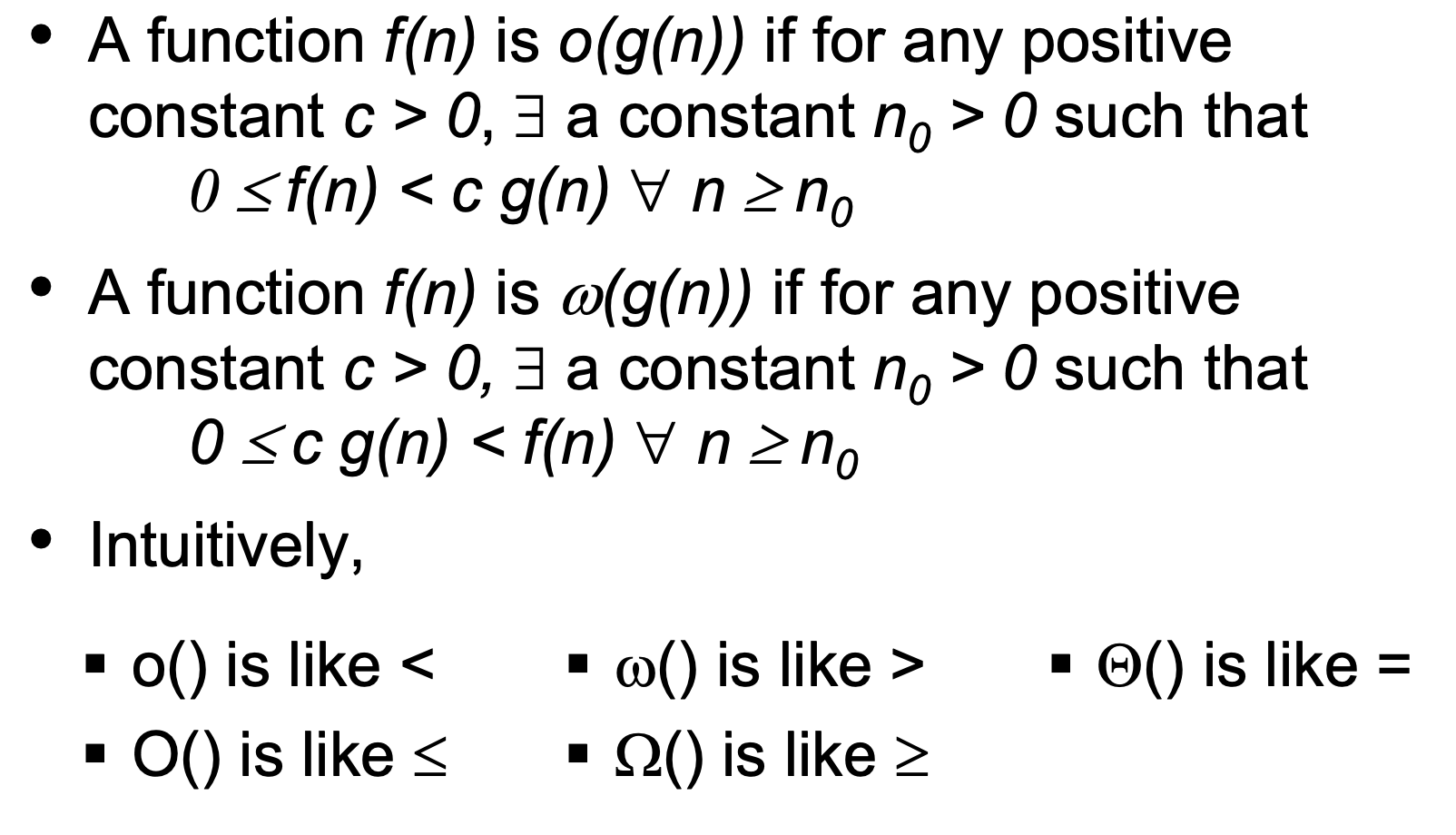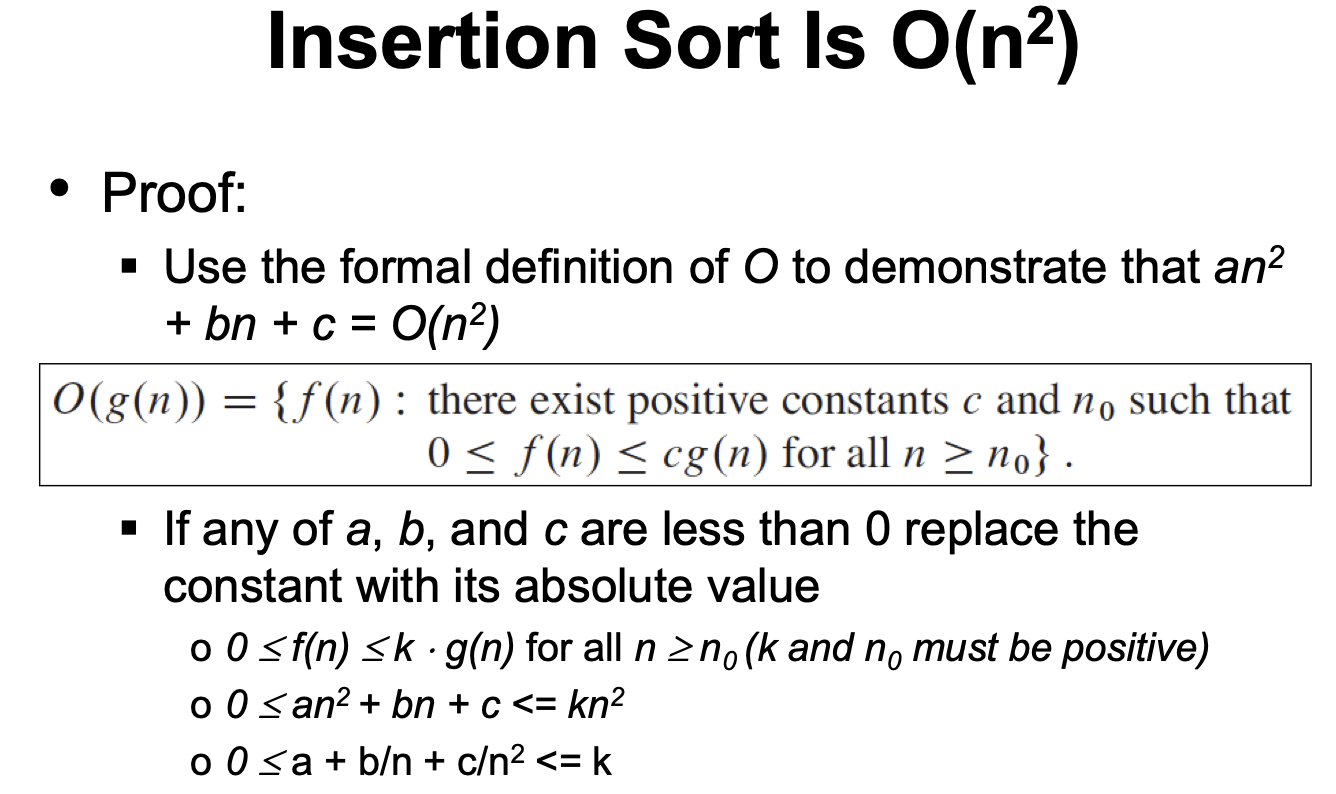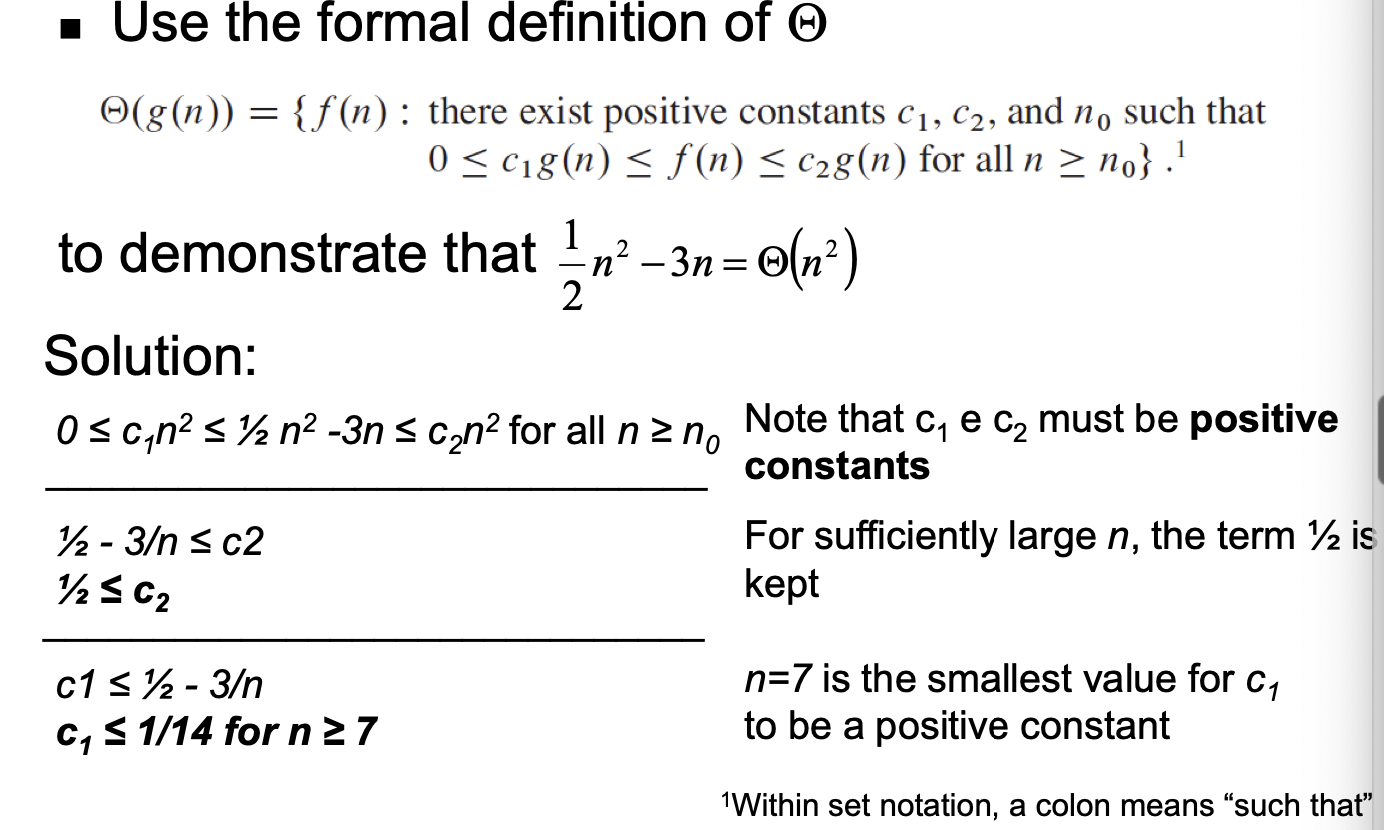### 1.2 分治

1. 分划 (Divide): 将原问题拆分成规模更小的子问题.
2. 解决 (Conquer): 递归地解决这些拆分出来的小问题.
3. 联合 (Combine): 将解决的小问题联合从而形成对原问题的一个解.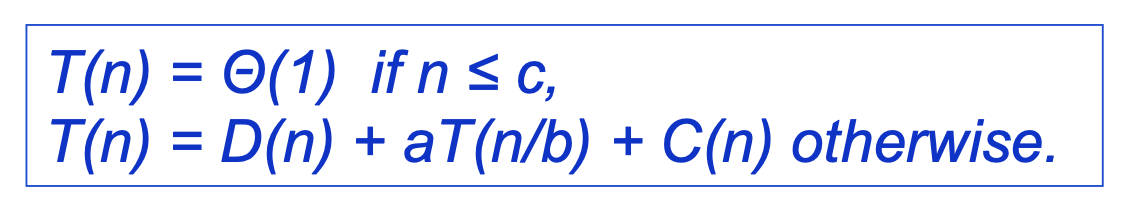1
2
3
4
5
6
7
8
9
10
11
12
13
14
15
16
17
18
19
20
21
22
23
24
25
26
27
28
29
30
31
MergeSort(A, l, r) {
if (l < r) {
mid = l + (r-l)/2;
MergeSort(A, l, mid);
MergeSort(A, mid+1, r);
Merge(A, l, r, mid);
}
}

Merge(A, l, r, mid) {
// take 2 sorted subarrays of A and merge them into 1 single sorted array
n1 = mid-l+1
n2 = r-mid

for (i=0; i<n1; i++) {
L[i] = A[l+i];
}
for (j=0; j<n2; j++) {
R[j] = A[mid+1+j];
}
L[n1], R[n2] = +infty;
i, j=0;
for (k=l; k<r+1; k++) {
if (L[i] <= R[i]) {
A[k] = L[i++];
} else {
A[k] = R[j++];
}
}

}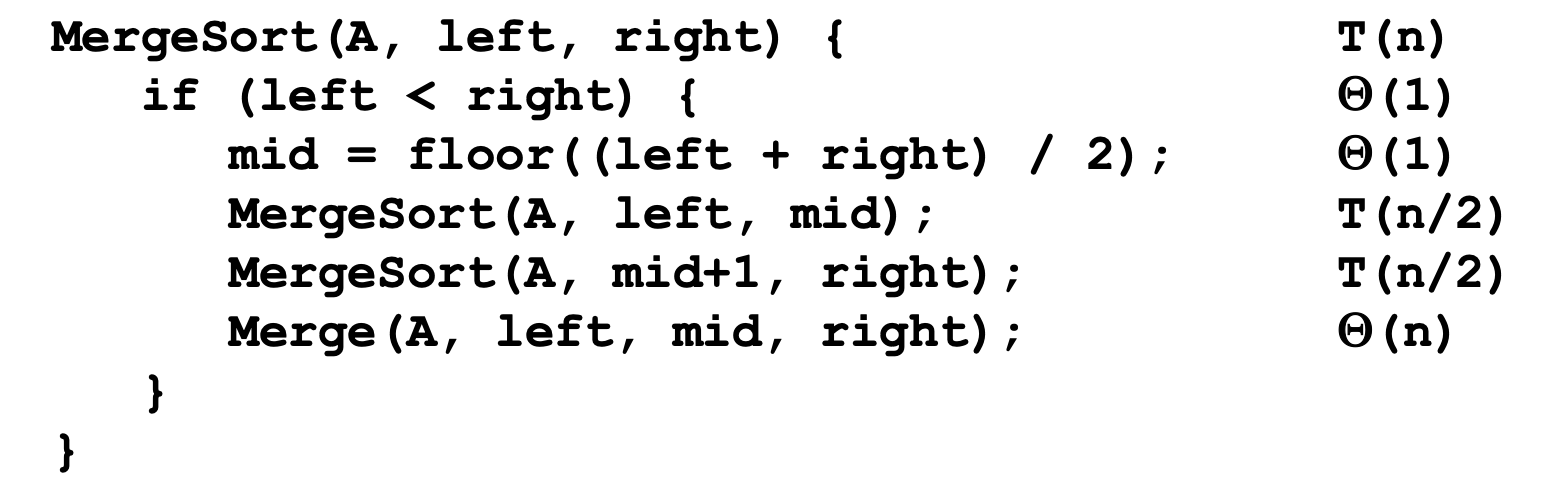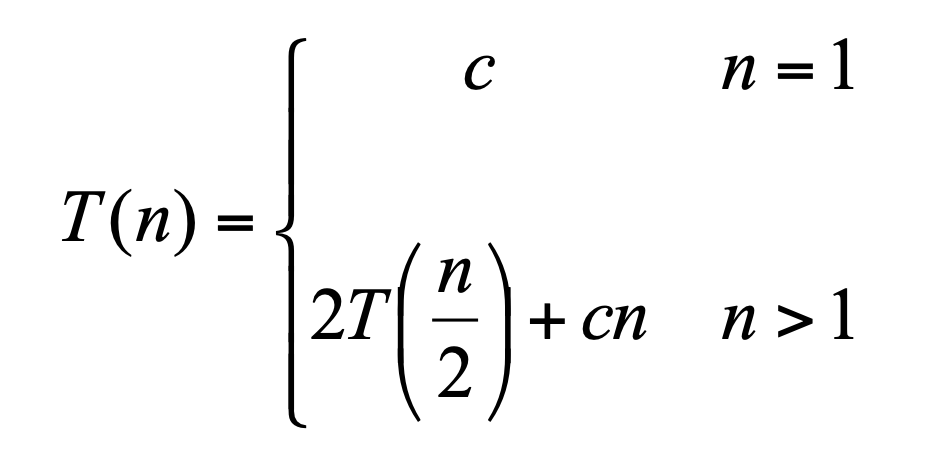1
2
3
4
5
6
7
8
9
10
11
12
BinarySearch(A, target) {
if (A.length == 1) {
return A == target;
}

mid = A.length/2;
if (target < A[mid]) {
BinarySearch(A[0 : mid-1], q);
} else {
BinarySearch(A[mid :], q);
}
}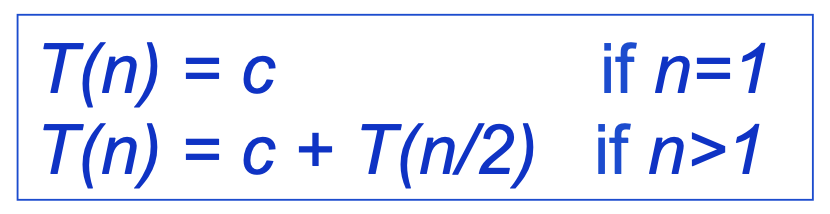### 1.3 求解递归表达式

1. 替代法 (Substitution Method): 基本原理是 猜测正确答案的形式 (猜测给定递归表达式的时间复杂度), 然后使用 数学归纳法 (Induction) 证明这个猜测成立.

值得注意的是, 在替换法中, 有时我们可以使用适当的技巧简化问题. 如: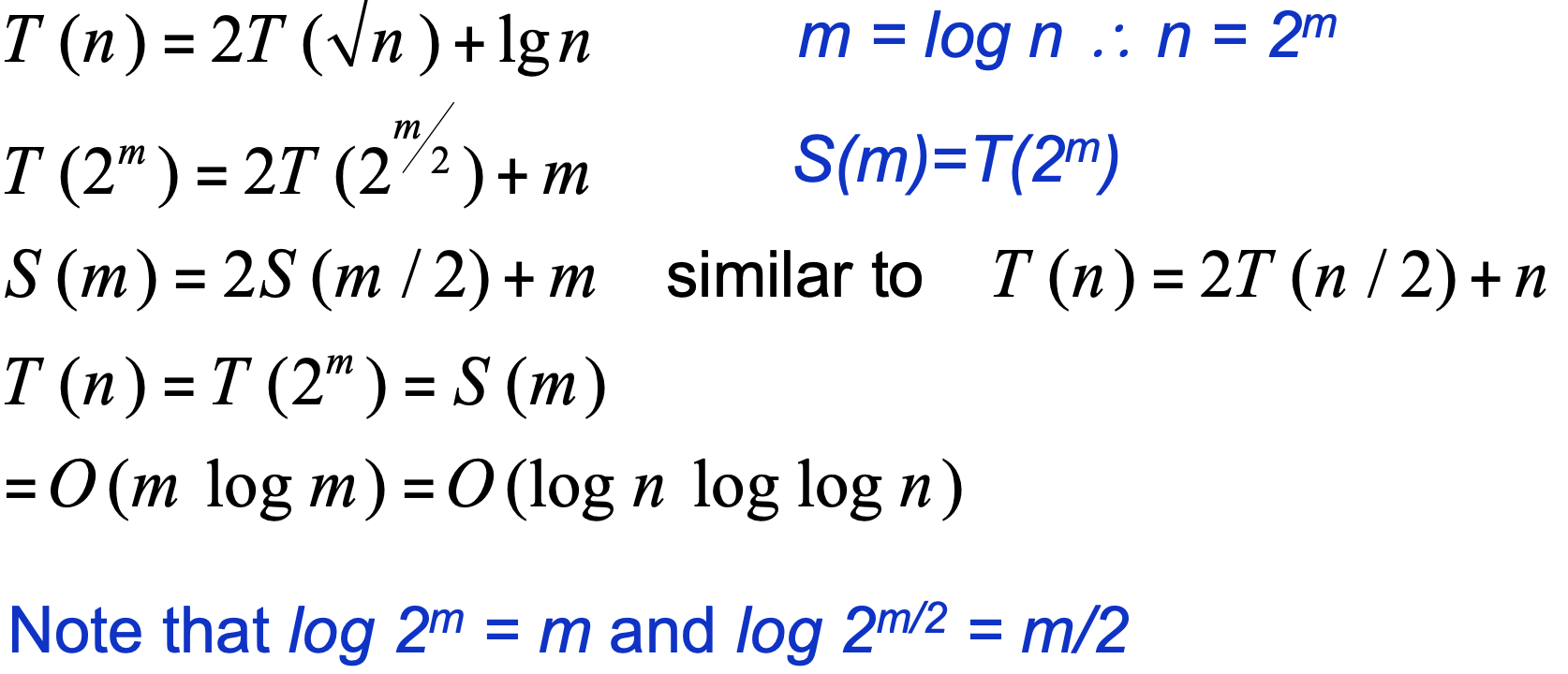在上面的例子中, 我们 通过将递归表达式右侧式子中的函数项和加号右侧的代数项替换为正常形式, 成功地将问题转换为我们可以解决的形式.

而在考虑 “将什么变量替换成什么形式” 的问题时, 可以优先考虑将等号右侧加号右边的项替换为一个 一次项, 如将 $\log(n)$ 替换为 $m$. 在此基础上, 再检查经过这样替换后的递归公式是不是被简化成了我们已知的一些常见形式. 如果是的话, 就可以使用替代法求解.

我们再附上一些指数, 对数, 阶乘和级数的常用性质: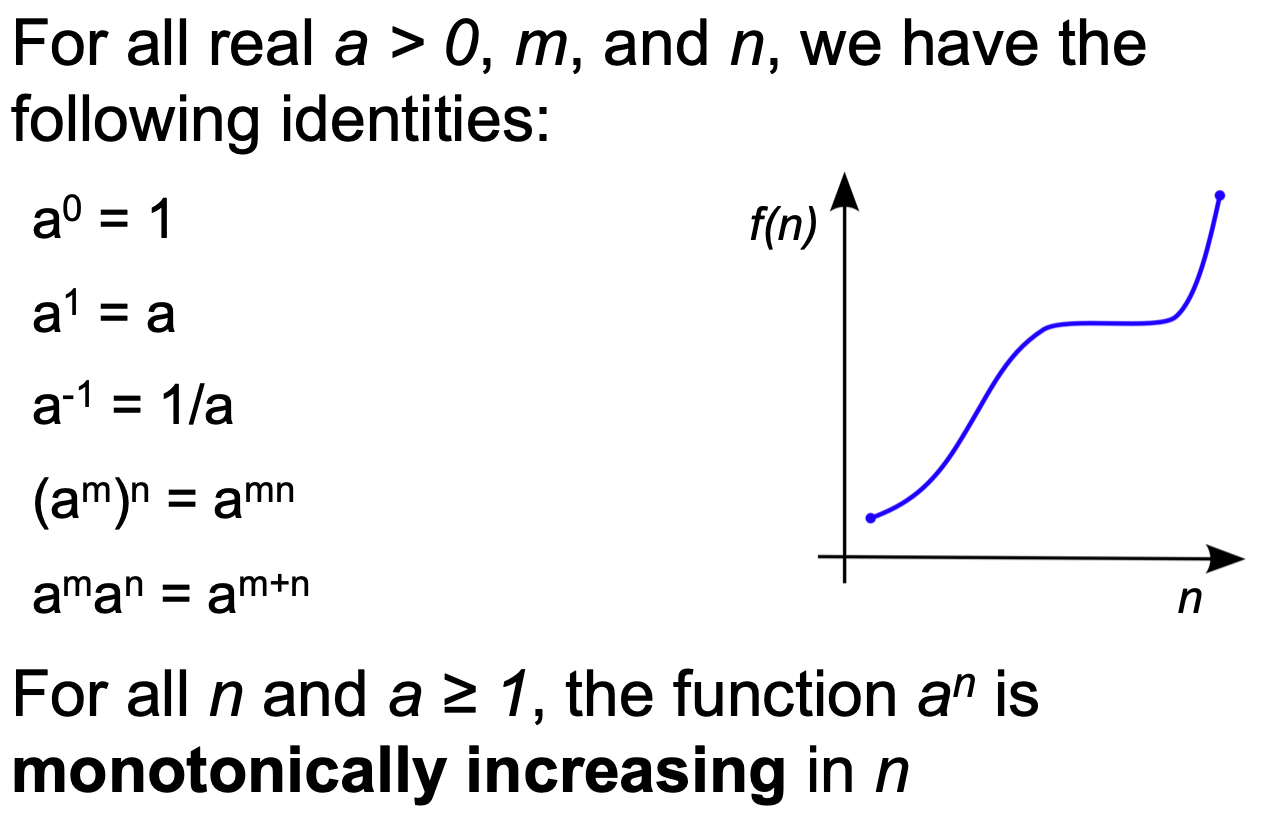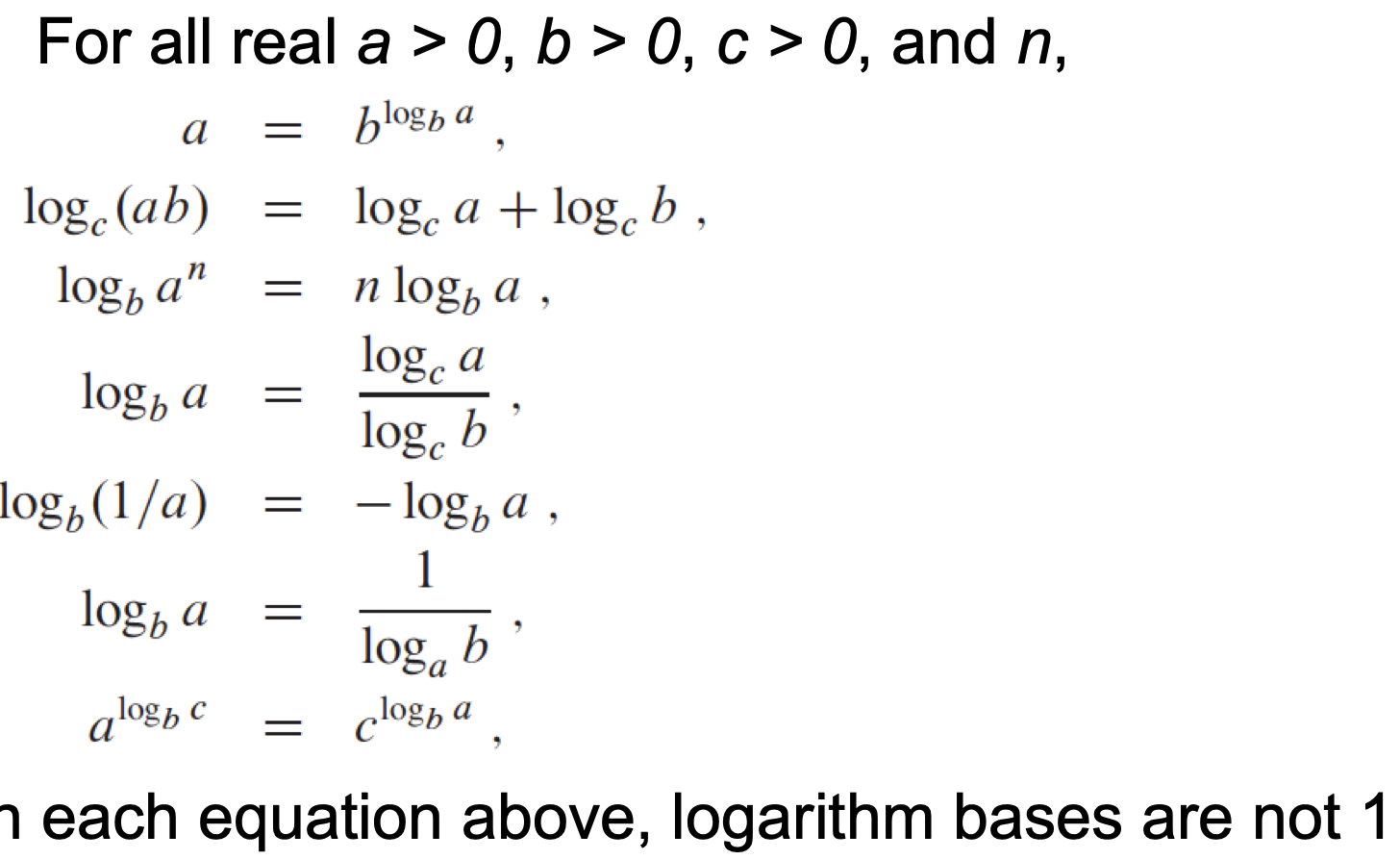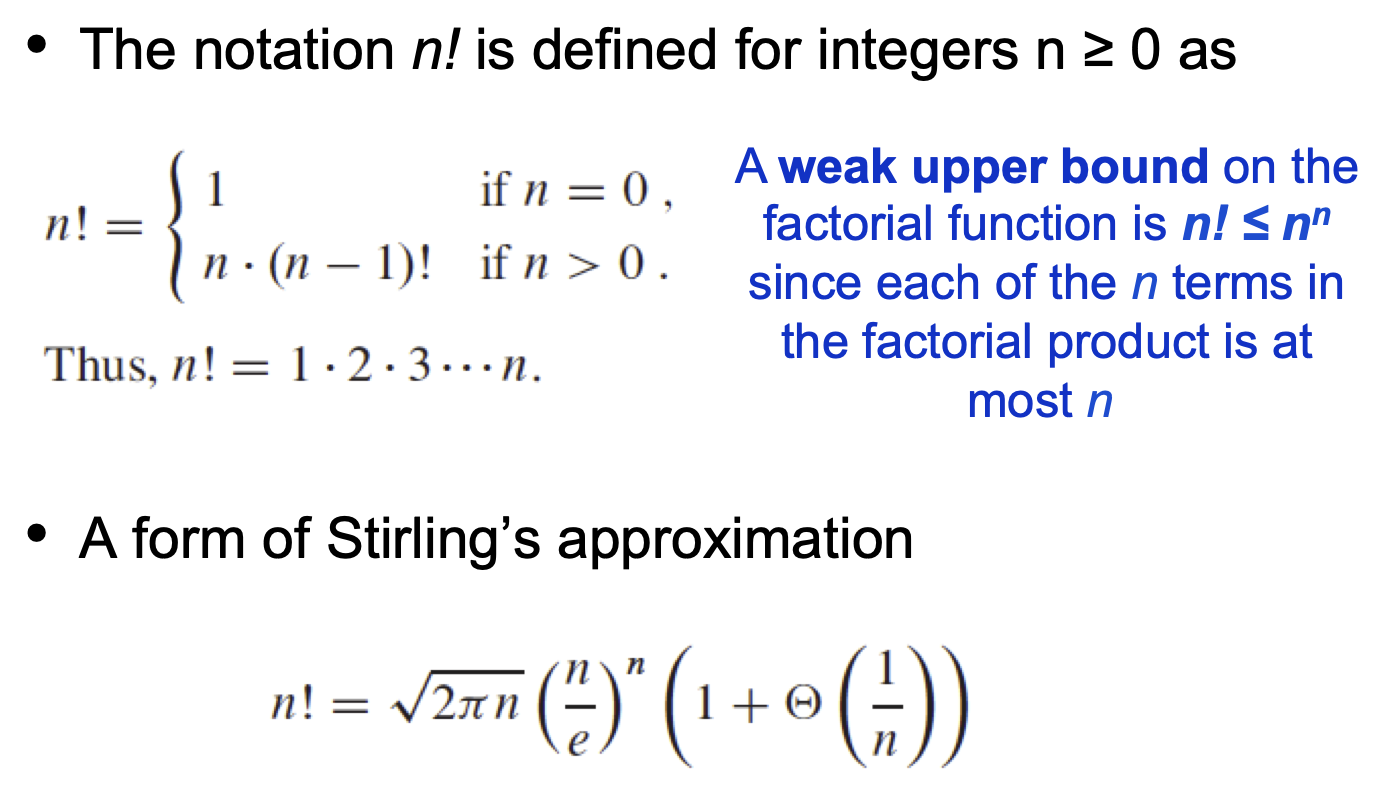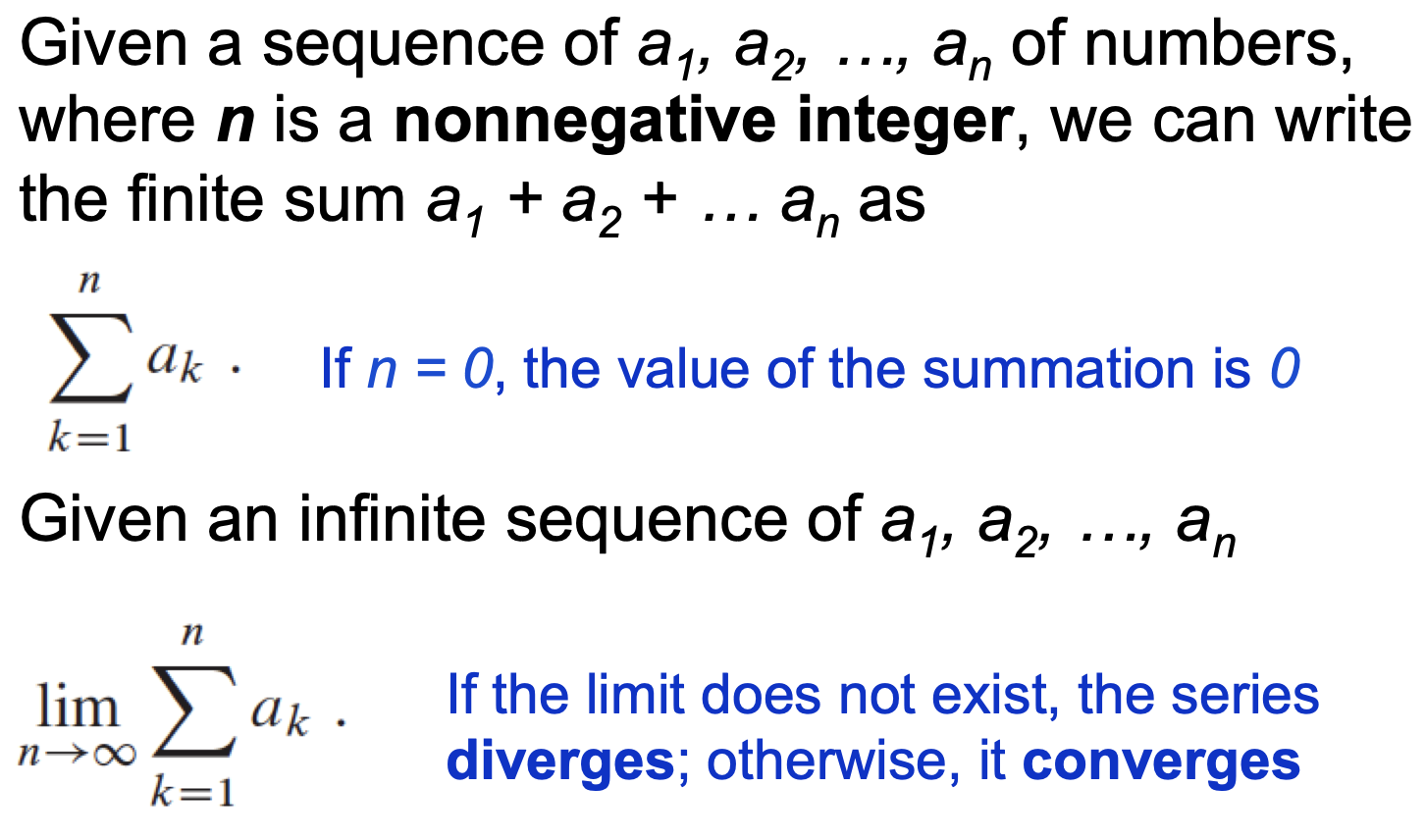此外, 我们还可使用定积分近似某个求和表达式: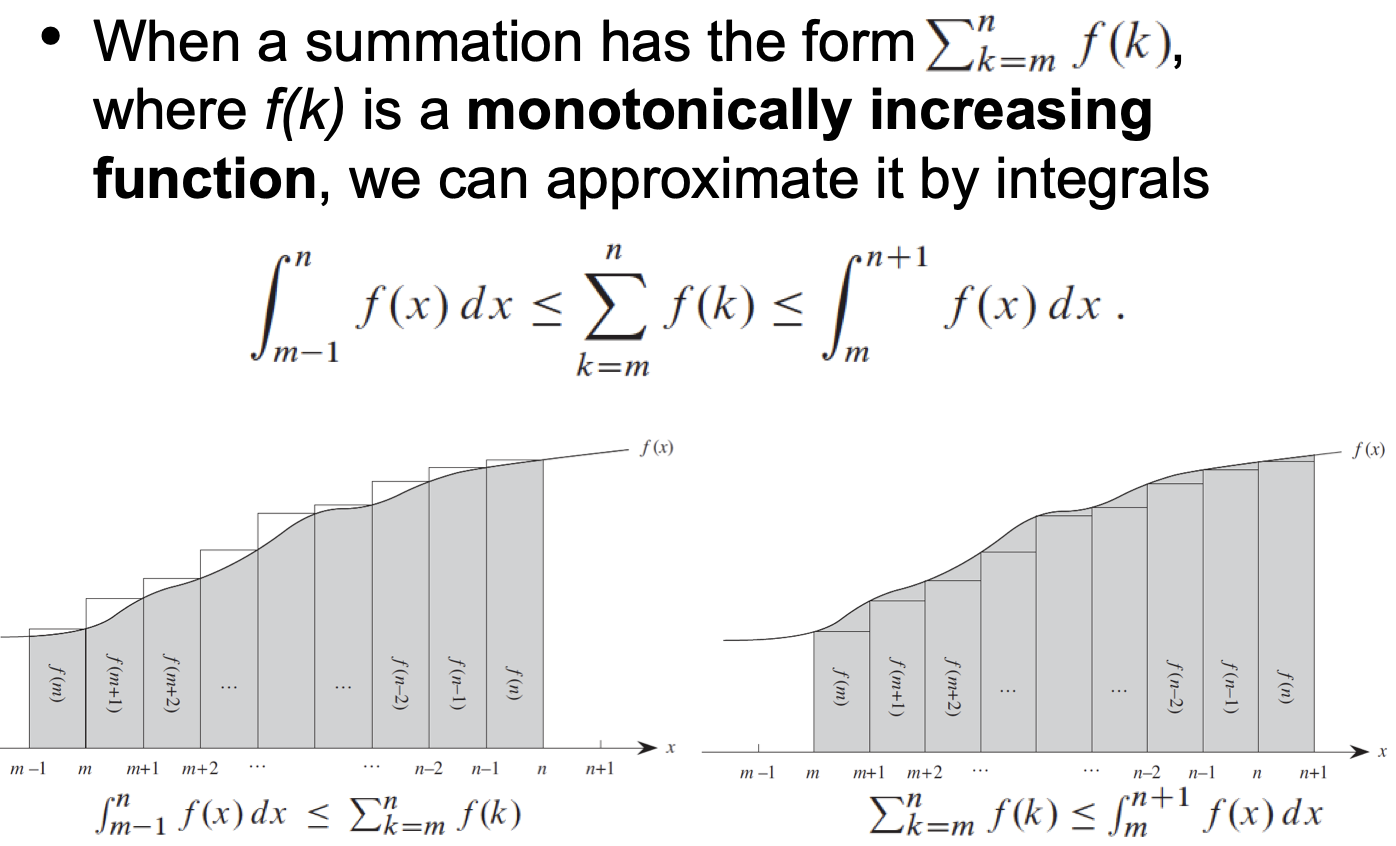2. 递归法 (Iteration Method) 递归法的基本原理是: 给定某个算法的递归表达式, 利用表达式自身循环定义的特性, 不断地将等式右侧的表达式使用递归定义 “解压缩”, 直到展现出某个明显的规律为止.

然后, 尝试将等号右侧已经表现出一定规律的式子中左边函数定义的那部分中的变量进行替换或变形, 从而使该变量等于函数递归表达式定义中 Base Case 对应的变量值. 这样, 我们就可以将函数定义的那部分直接替换为 Base Case 对应的式子 (但一般都是个数值), 从而可以直接看出表达式的时间复杂度.

举例: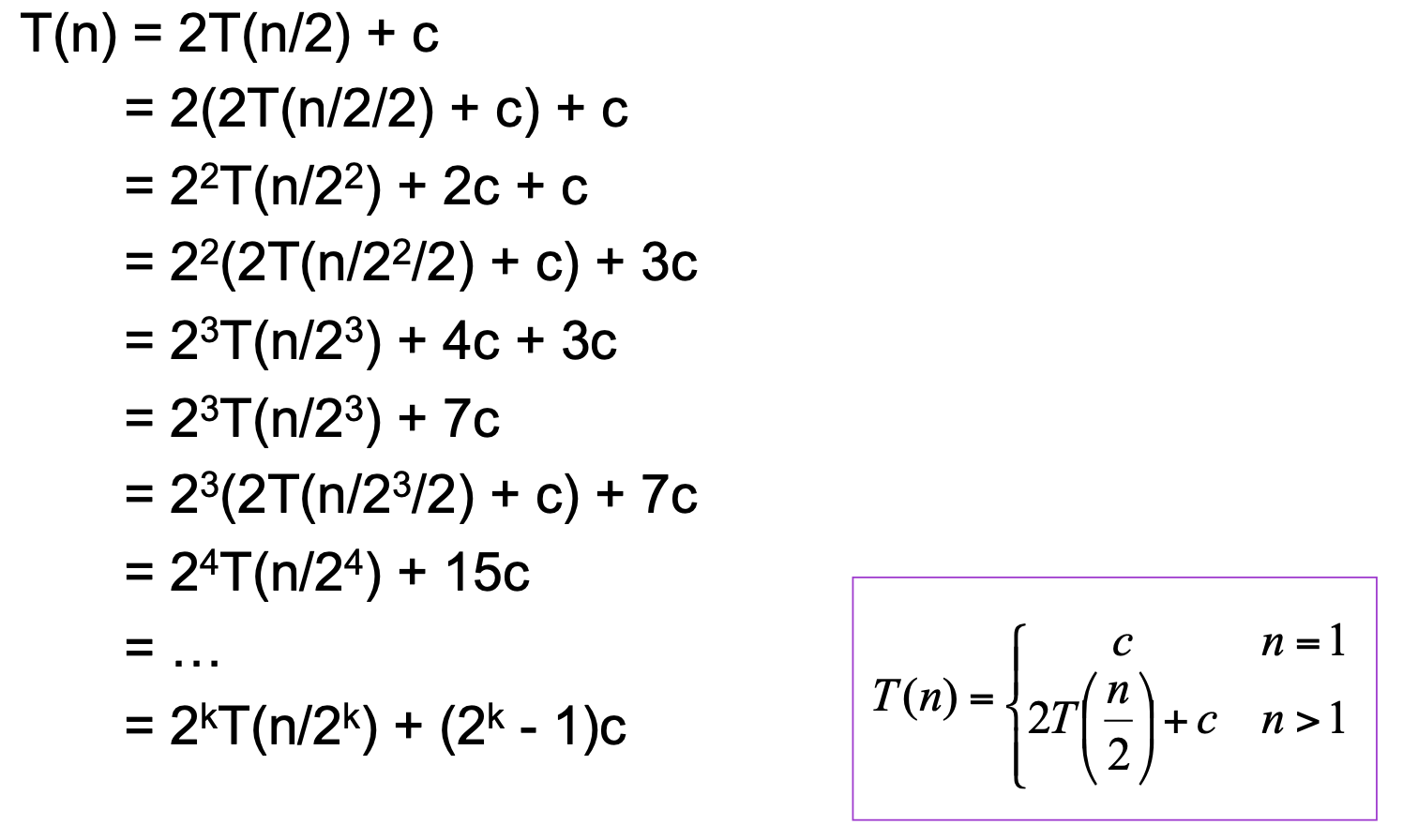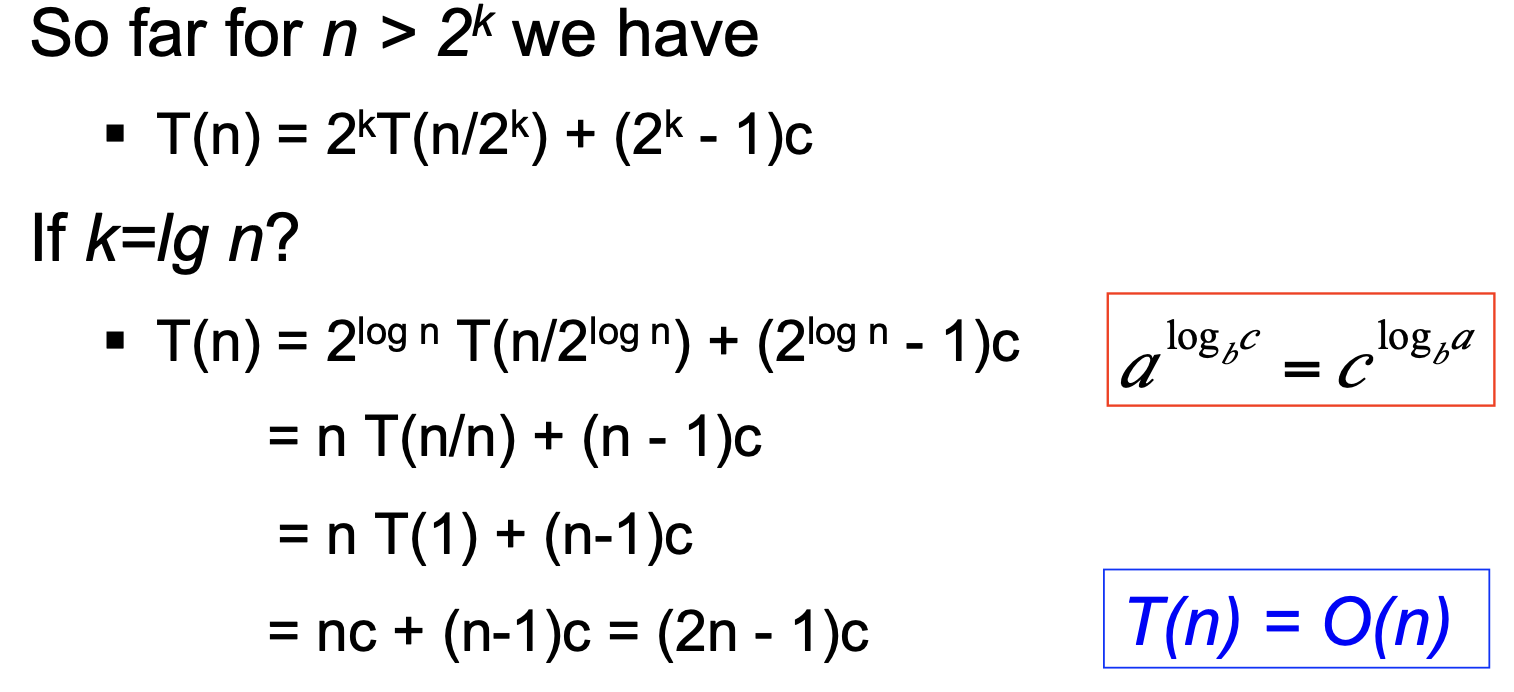3. 使用主定理 Master Theorem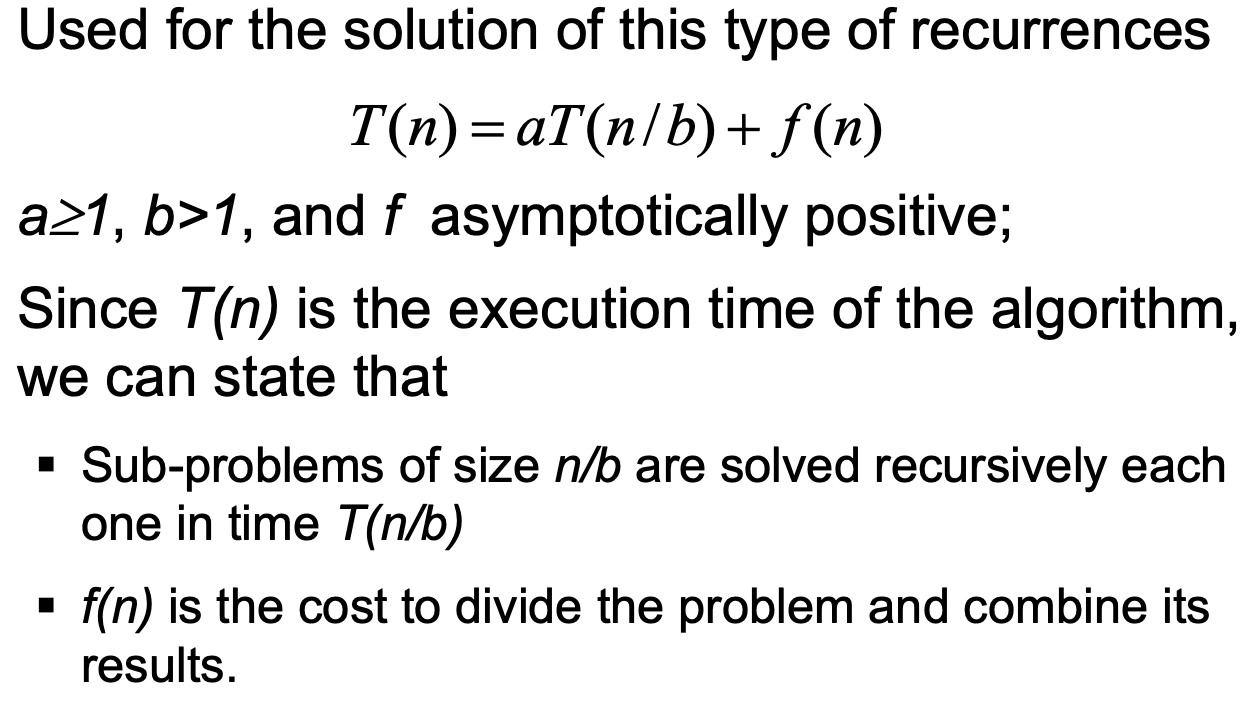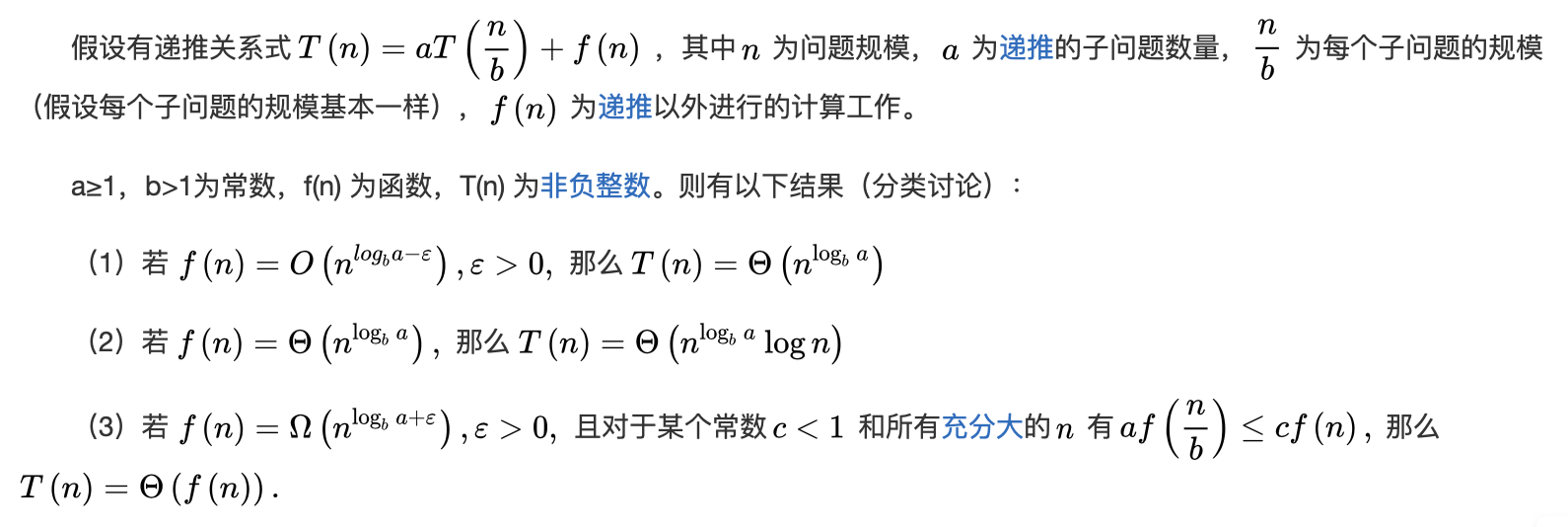下面给出一些常用结论:

\begin{aligned} T(n) &= T(n-1) + O(1) ~~~ \rightarrow \Theta(n) \\ T(n) &= 2T(\frac{n}{2}) + O(1) ~~~~~~~\rightarrow \Theta(n) \\ T(n) &= T(\frac{n}{2}) + O(1) ~~~~~~~~~\rightarrow \Theta(\log(n)) \\ T(n) &= T(\frac{n}{2}) + O(n) ~~~~~~~~~\rightarrow \Theta(n\log(n)) \end{aligned}

### 1.4 算法性能的均摊分析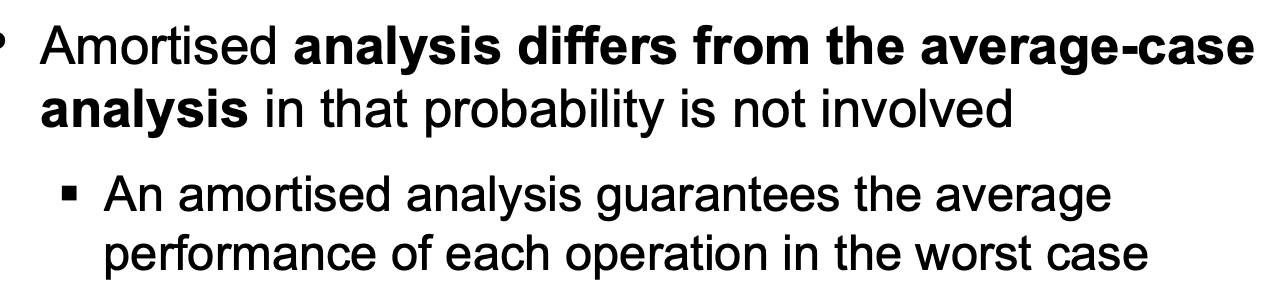1. Aggregate Method 聚合法.
2. Accounting Method 审计法.
3. Potential Method 势能法.

(后面提到的, 关于动态增删数组, 栈操作和二进制加减法的例子建议直接看 Slides, 此处省略)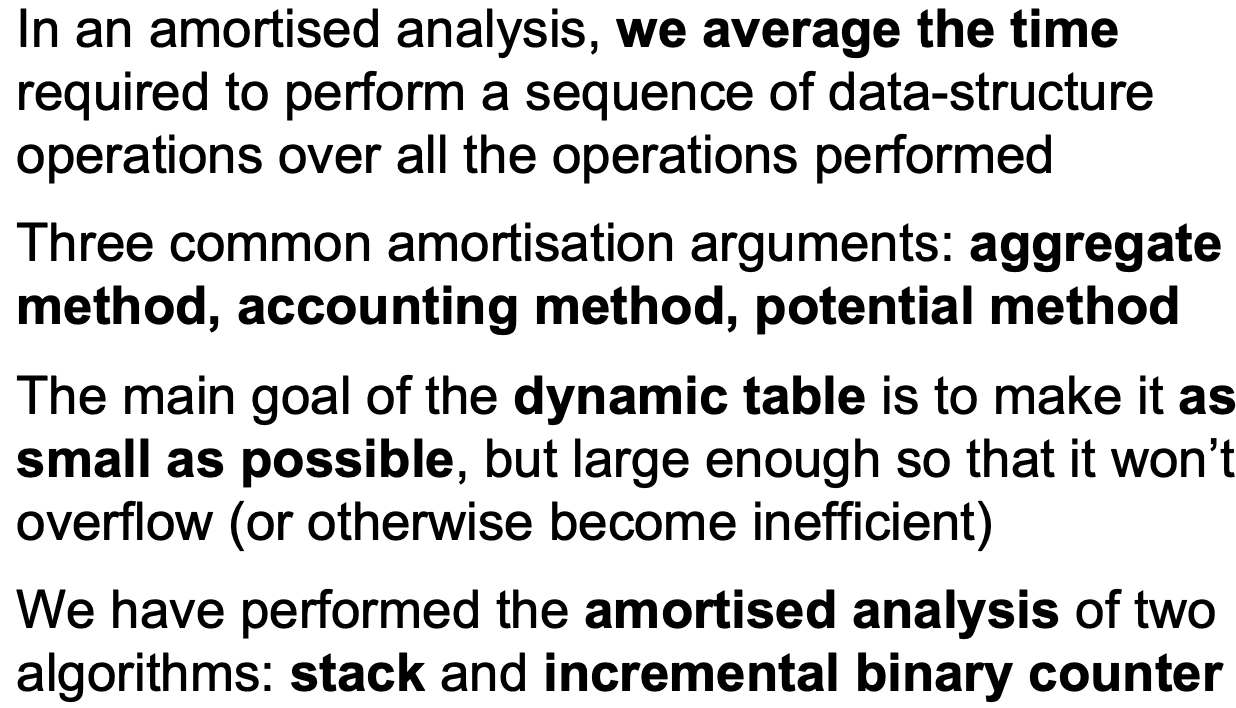## 2. 基本数据结构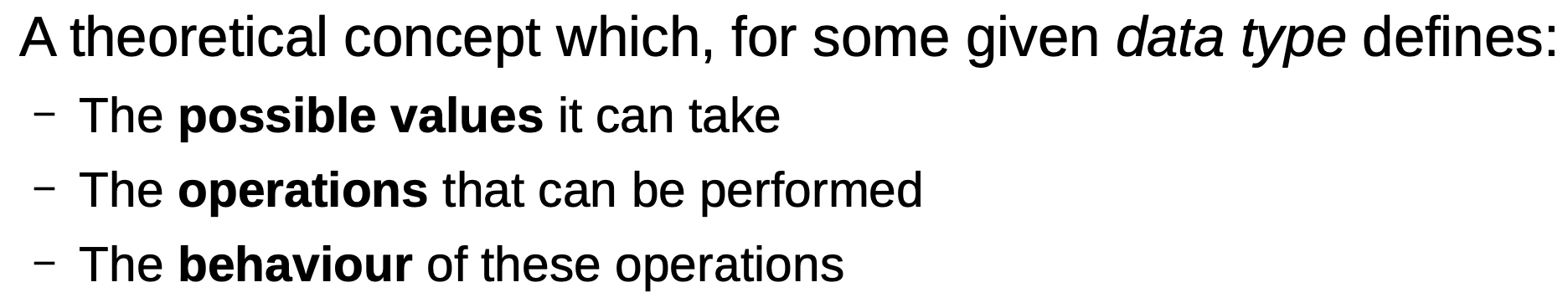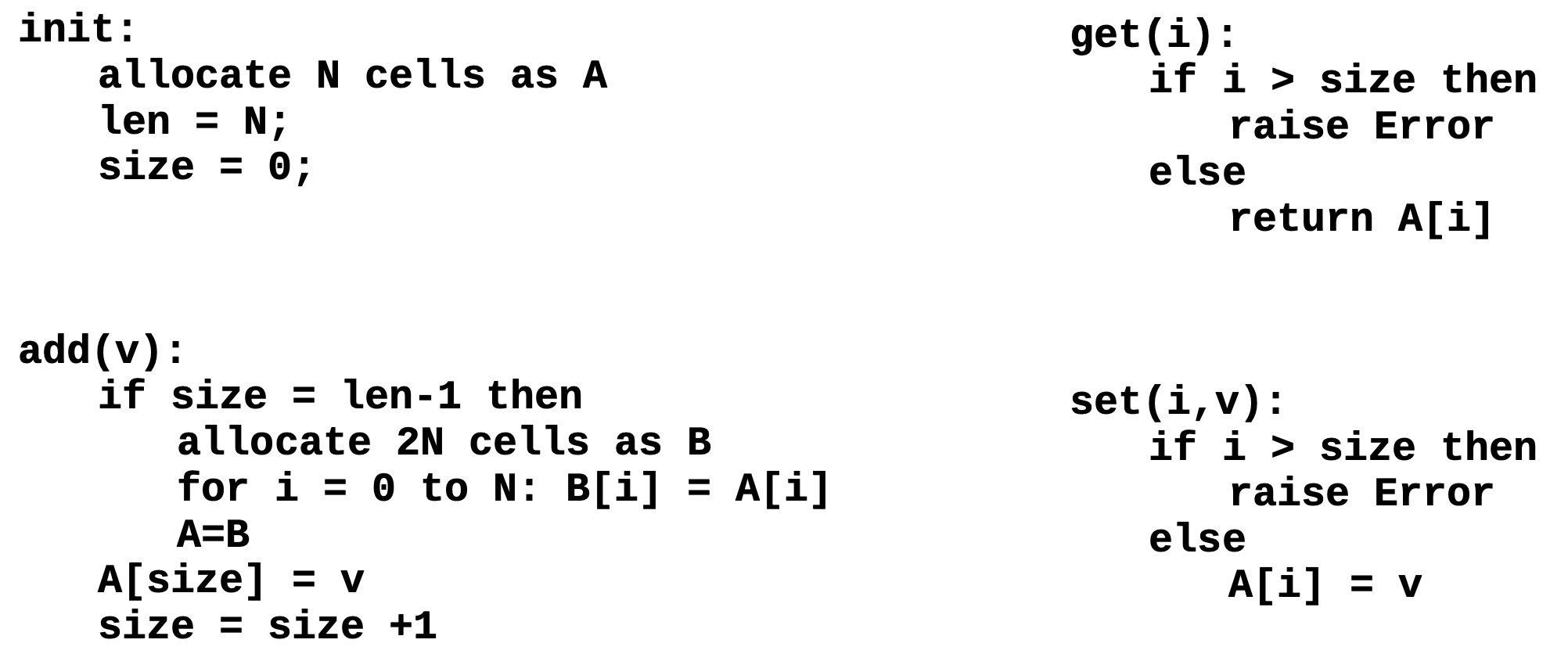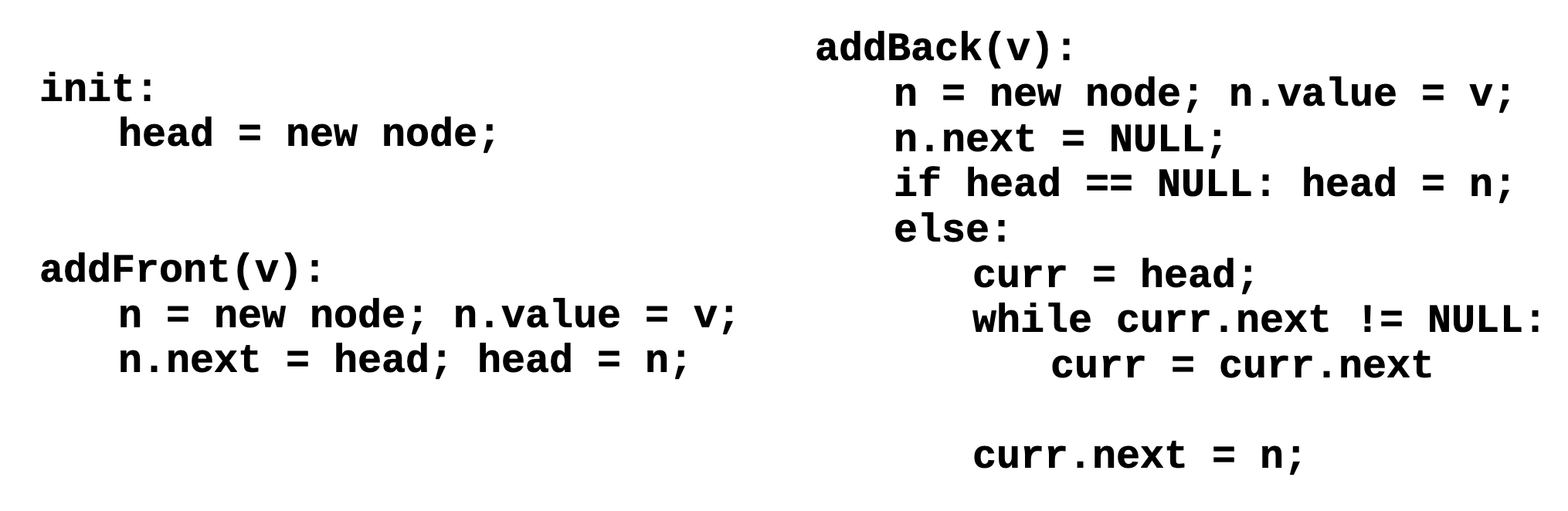### 2.1 哈希表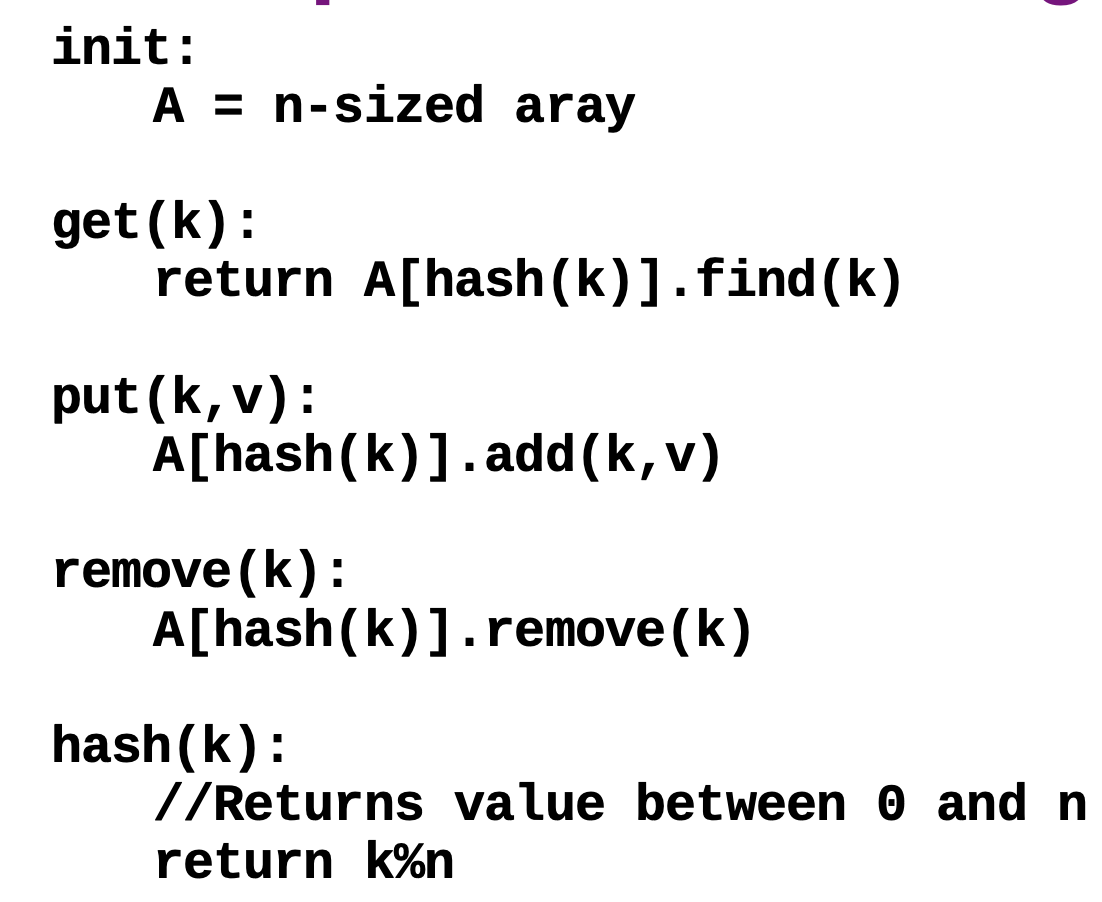1. 在将数据插入表中时, 使用经过精心设计的 哈希函数, 基于被插入数据本身的特性得到一个索引, 然后将数据存到实际负责数据存储的数组的索引位置上.
2. 如果数组的索引位置上已经有数据存储, 也就是发生了 “数据碰撞” (Collision), 则需要再基于某些规则重新生成一个新的索引, 把数据存到别的位置上去从而避免碰撞.
3. 在从表中提取数据时, 同样需要使用哈希函数算出索引, 然后按图索骥从数组中找到所需要的数据.
4. 在实际负责数据存储的数组剩余空间不足时, 需要将其扩容并将原数组中存放的所有元素全部重哈希到更大的新表中.

1. 什么是合理的哈希函数?

合理的哈希函数应当具有以下性质:

给定一个映射范围 $n$ 和被映射元素集合 $U$, 哈希函数 $f$ 应该能将 $U$ 中的每个元素 均匀地 映射到 $[0, n)$ 的范围上.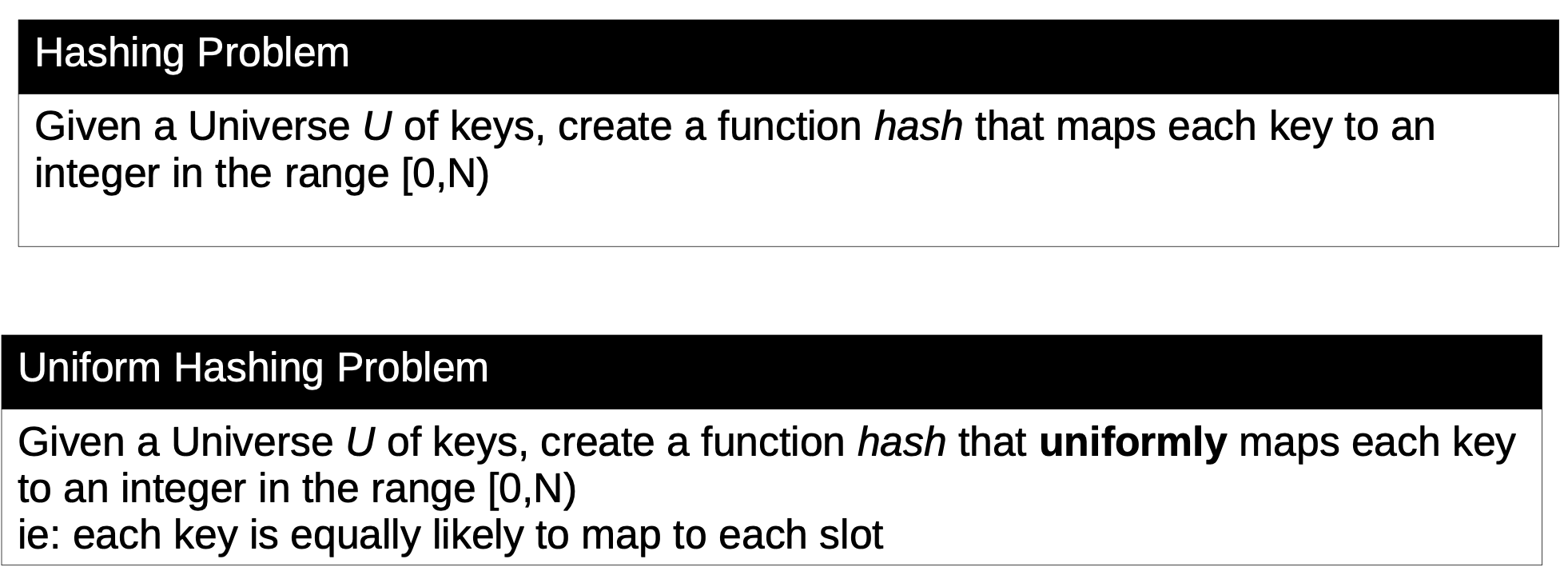能够正确区分性质相似的数据, 如包含相同元素而排列顺序不同的字符串.

2. 我们无法避免数据碰撞的发生. 一旦发生数据碰撞, 有哪些可行的方法解除数据碰撞?
• Separate Chaining: 若多个数据被哈希函数映射到数组的同一个位置上, 则在数组的该位置上存储一个链表, 将这些数据按照插入的先后顺序挂到链表上.

在实际应用中, 只要哈希函数的选取和重哈希策略得当, 在数组中即使存在数据碰撞, 该位置上的链表也不会很长.

• Open Addressing: 开放寻址, 在确定出现数据碰撞后使用预定义的规则再生成新的位置, 直到生成的新位置上不存在数据碰撞为止.

在实际应用中, 如果规则定义不当, 就容易在哈希表中出现数据堆积的情况.

开放寻址的实现方式一般又有三种: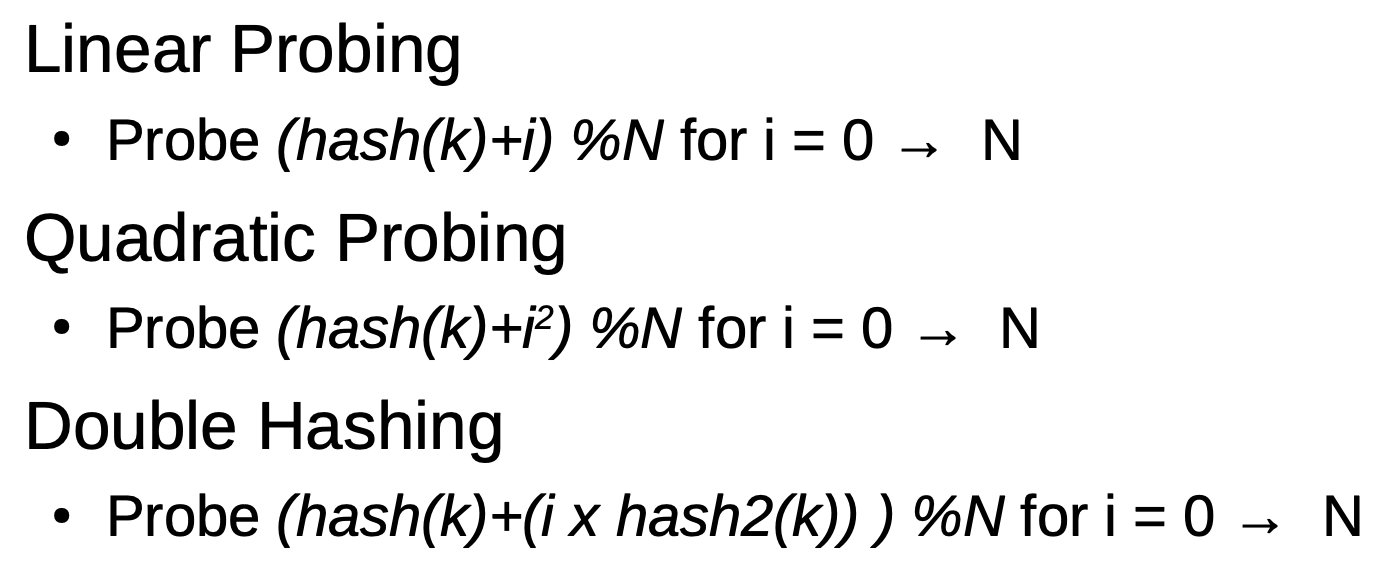3. 重哈希的策略是什么? 何时需要重哈希?

重哈希的策略是: 首先调整在哈希函数中涉及到的变量: 存储数组的预期大小, 然后新建一个大小为预期尺寸的, 比原数组更大的空数组.

然后, 基于这个 修改过的哈希函数 (哈希函数中取模时依赖的, 存储数组的预期大小变大了) 将原数组中的所有元素全部重哈希到新数组中.

丢掉原数组, 将更大的新数组视为哈希表的存储数组.

我们使用反映哈希表的存储数组的利用率的变量 负载常数 (Load Factor) 检测哈希表的使用率, 从而控制何时执行重哈希.

一般而言, 我们会选定重哈希阈值为 $0.75$.

如果阈值选定不当, 会导致哈希表的存储数组中可用的剩余空间过少, 以至于在查询和插入数据时哈希函数的数据碰撞次数显著增大, 在最坏情况下对哈希表的搜索操作会退化为线性搜索, 而在一般情况下搜索的时间复杂度仅为 $O(1)$.

### 2.2 搜索树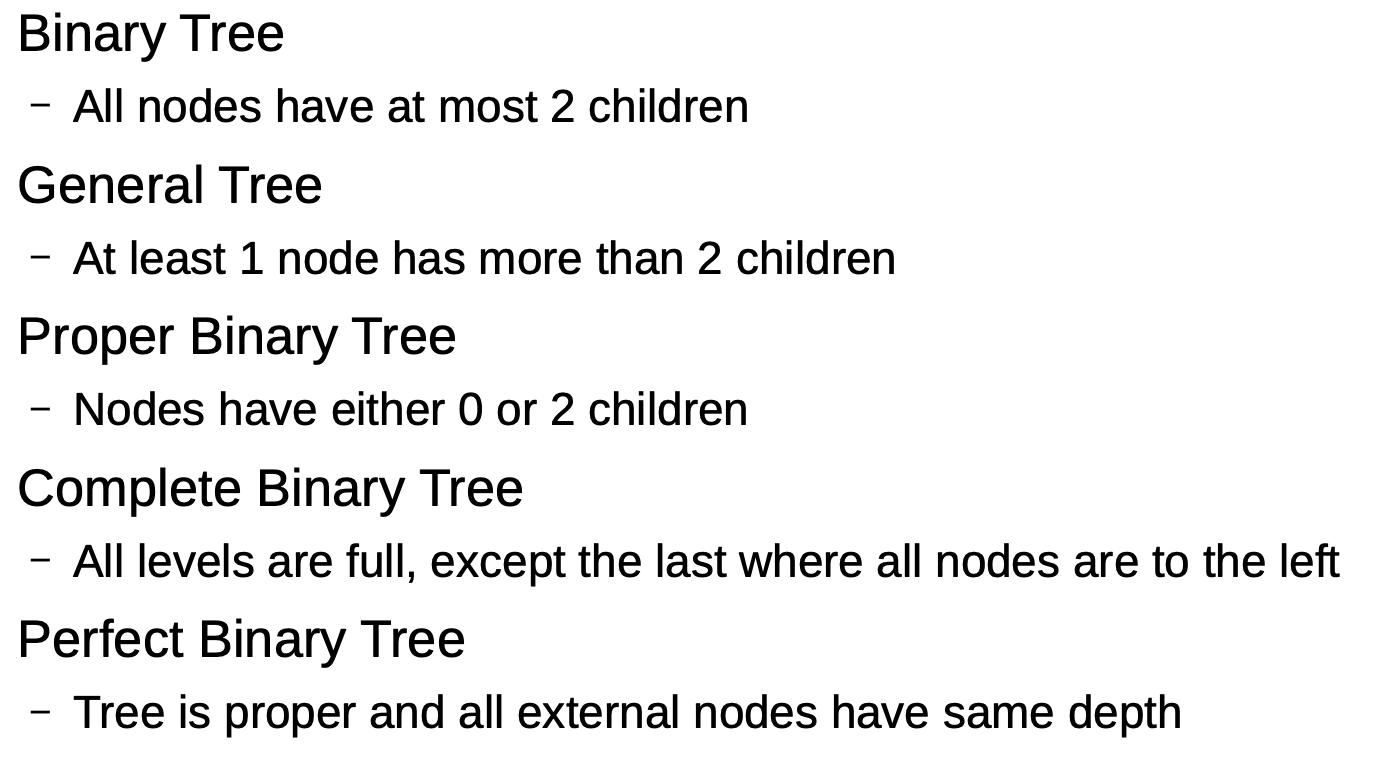1. Proper Binary Tree/Full Binary Tree (满二叉树) $\Rightarrow$ 每个节点的度或为 $0$ 或为 $2$.
2. Complete Binary Tree (完全二叉树) $\Rightarrow$ 叶子结点只出现在 最下层或次下层, 且 最下层的叶子结点集中出现在树的左部 (除了最后一层外, 其他各层节点数都达到最大, 且最后一层的节点都连续集中在最左边).
3. Perfect Binary Tree (完美二叉树) $\Rightarrow$ 在满二叉树的基础上, 所有叶子结点深度均相同.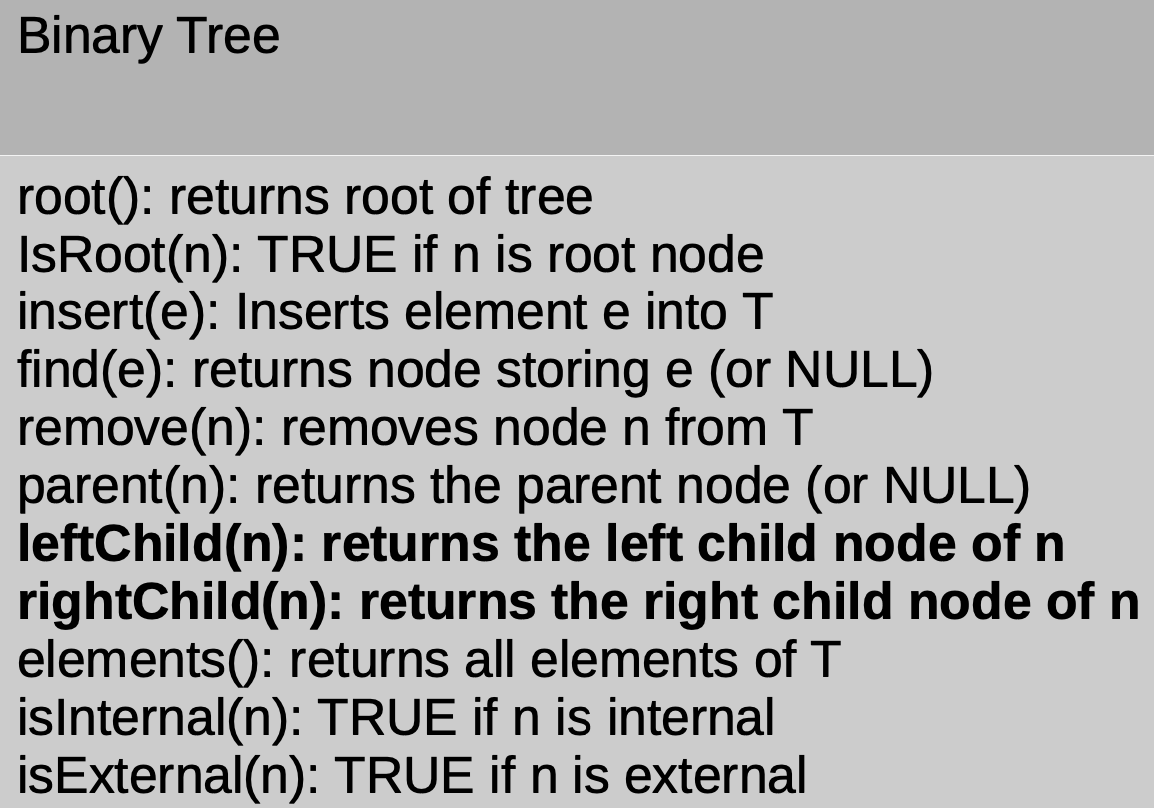1. 二叉树的 外部节点 (Exterior Node) 指他的叶子结点.
2. 二叉树中结点所拥有的子树个数称为结点的 (Degree).
3. 二叉树的 高度 为树中所有节点深度的最大值, 也就是从叶子结点到根节点所需要经过的边的 最大值, 从 $0$ 起算.
4. 二叉树中节点的深度为从该节点到树的根节点所需经过边的数量, 也是从 $0$ 起算.

1. 二叉树节点的插入: 只需基于给定的被插入值大小沿着树遍历, 直到在保持不变量基础上, 左子节点 (或对应的, 右子节点) 为空的节点, 然后将新节点作为该节点的左子节点/右子节点插入即可.

2. 二叉树节点的删除:

• case 1: 若要被删除的结点为叶子结点或左右子节点均为空, 则直接删除节点的父节点中, 对应指向该节点的指针.
• case 2: 若要被删除的节点有一个子节点 (左子节点或右子节点), 则将该节点的父节点中, 对应的指针指向它的子节点即可.
• case 3: 若要被删除的节点有两个子节点, 就需要从这个被删除节点开始执行 中序遍历 来找到它的 后继节点 $p$ (一般是左子树中的最大值所在的节点或右子树的最小值所在的节点).

然后将被删除节点和 $p$ 的值调换, 递归地执行对节点 $p$ 的删除操作, 这也是删除根节点所对应的状况:

1
2
3
4
5
6
7
8
9
10
11
12
13
14
15
16
17
18
19
20
21
22
23
24
25
26
27
28
29
30
31
TreeNode delNode(TreeNode root, int key) {

// 先遍历到目标节点位置上, 若找不到目标节点则直接返回当前所在节点不改动树
if (! root) {
return root;
} else if (root.val > key) {
root.left = delNode(root.left, key);
} else if (root.val < key) {
root.right = delNode(root.right, key);
}

// 定位到节点后开始删除
else {
// case 1, 2: 直接删除, 子树提升一级
if (root.left == null || root right == null) {
root = root.left? root.left: root.right;
} else {
// case 3: 找到右子树中被删除节点的后继, 交换值后递归删除后继
TreeNode current = root.right;
// 中序遍历找后继
while (current.right) {
current = current.left;
}
// 交换节点值
root.val = current.val;
// (递归) 删除后继, 或者后继的后继, ....
root.right = delNode(root.right, root.val);
}
}
return root;
}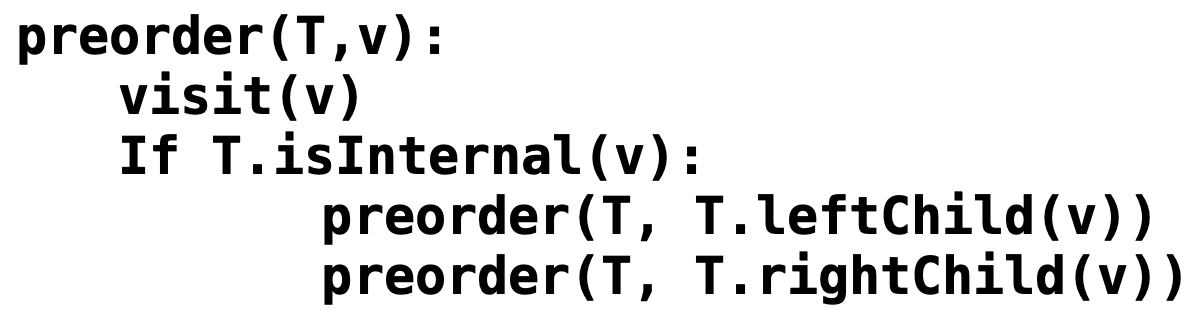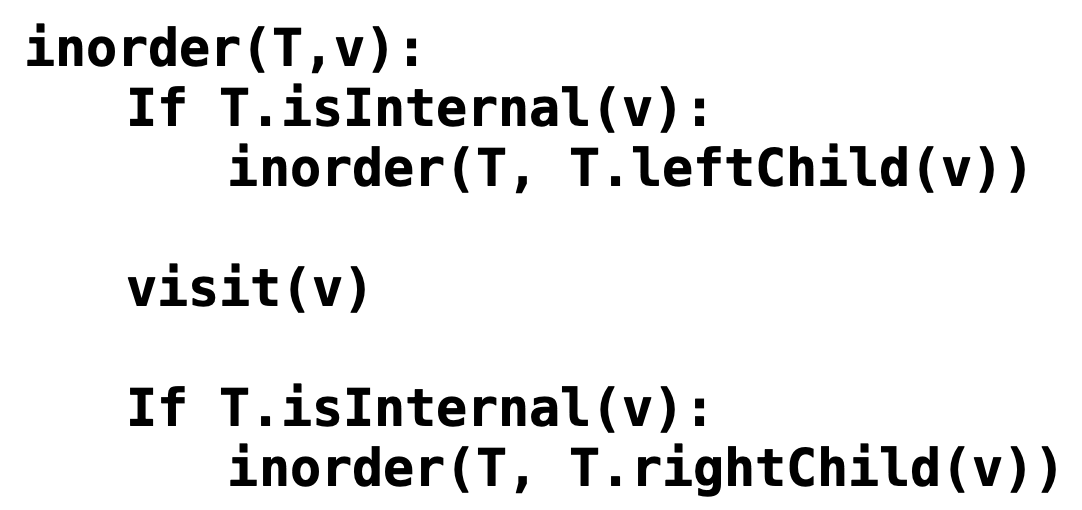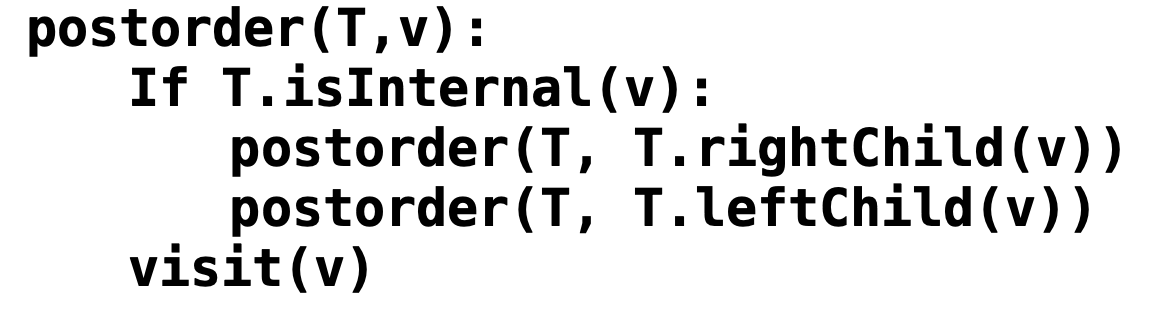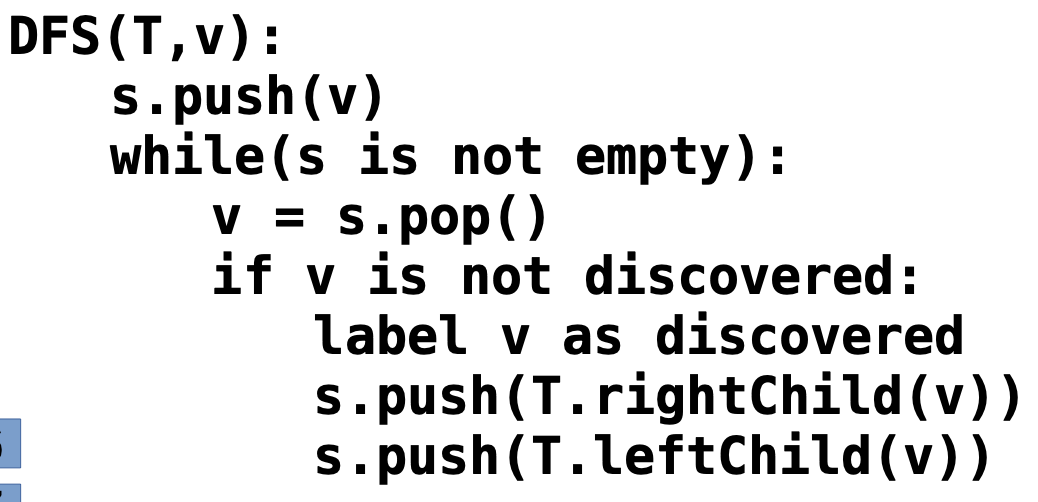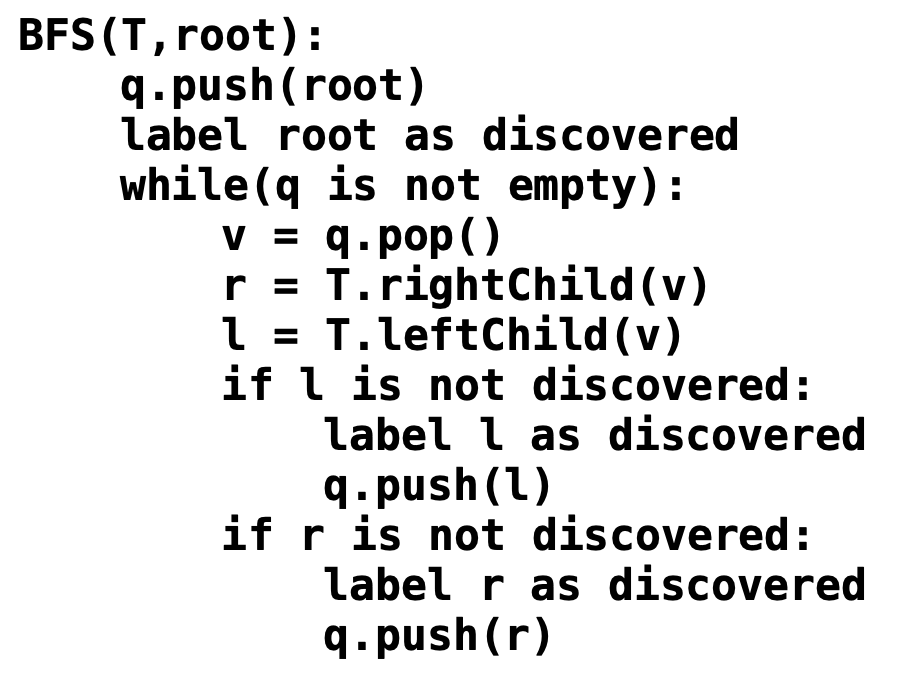AVL 树 (自平衡二叉树) 是为了解决这一问题而设计出的数据结构. 其主要特征 (也是解决二叉树不平衡导致的性能衰减问题的手段) 是, 它维护了另一个不变量: 节点的平衡度.

AVL 树中, 节点的平衡度 被定义为: 该节点左右子树高度差的绝对值, 而 AVL 树相比二叉搜索树, 所维护的第二个不变量就是, 对树中的任何节点而言, 它的平衡度必须 小于等于 $1$, 该属性也被称为 高度平衡属性 (Height-Balance Property).

AVL 树的旋转分为 单旋转双旋转, 任何旋转操作都有两个属性: 旋转轴旋转方向. 旋转轴本质就是 旋转之后的子树的节点.

1. 左旋

如果观察到某个节点 不满足高度平衡属性 且它的 右子树 高于左子树, 则需要对该节点执行 左旋操作:

1. 将指向该节点的指针改为指向它的右子树.
2. 将该节点右子树改为它的 右子树的左子树: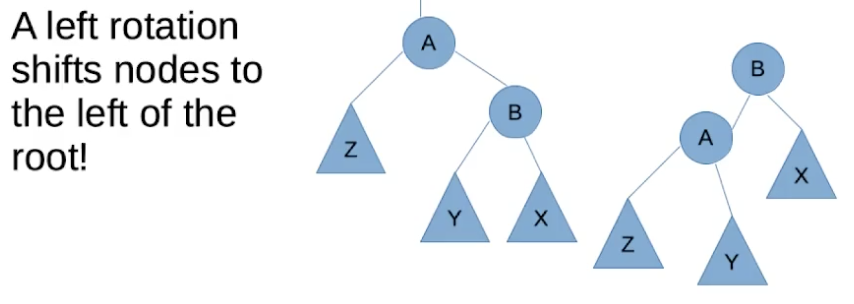在完成旋转操作后:

1. 根节点从原节点变为它的右子节点.
2. 原节点从根节点变为根节点的左子节点.
3. 根节点的右子节点的左子节点变为根节点的左子节点的右子节点.
2. 右旋

如果观察到某个节点 不满足高度平衡属性 且它的 左子树高于右子树, 则需要对该节点执行 右旋操作:

1. 将指向该节点的指针改为指向它的左子树.
2. 将该节点左子树改为它的 左子树的右子树: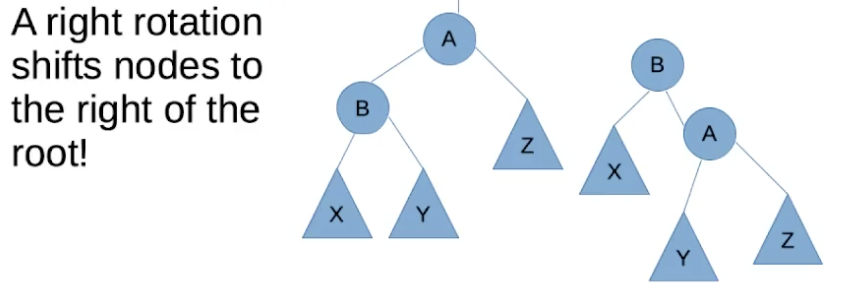在完成旋转操作后:

1. 根节点从原节点变为它的左子节点.
2. 原节点从根节点变为根节点的右子节点.
3. 根节点的左子节点的右子节点变为根节点的右子节点的左子节点.
3. 左-右旋

如果观察到某个节点 不满足高度平衡属性 且它的右子节点呈现出 “左重右轻” 的特点, 也就是该节点有一个 Left-heavy Right subtree, 则需要执行左-右旋: 先以右子节点为轴执行一次右旋让右子结点从 “左重右轻” 变成 “左轻右重”, 然后以原节点为轴执行一次左旋.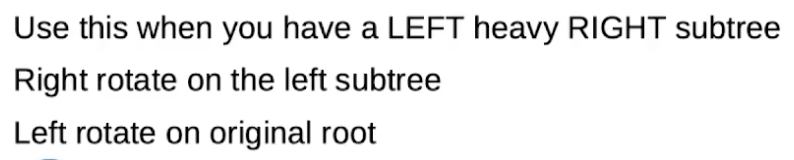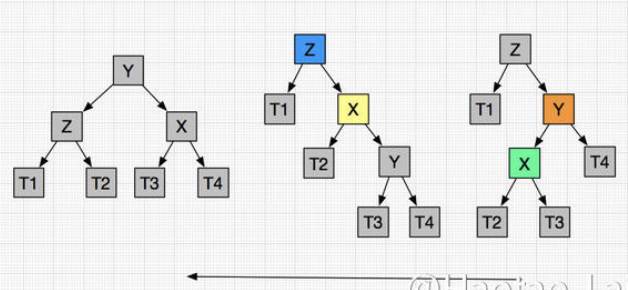4. 右-左旋

如果观察到某个节点 不满足高度平衡属性 且它的左子节点呈现出 “右重左轻” 的特点, 也就是该节点有一个 Right-heavy Left subtree, 则需要执行右-左旋: 先以左子节点为轴执行一次左旋让左子结点从 “右重左轻” 变成 “左重右轻”, 然后以原节点为轴执行一次右旋.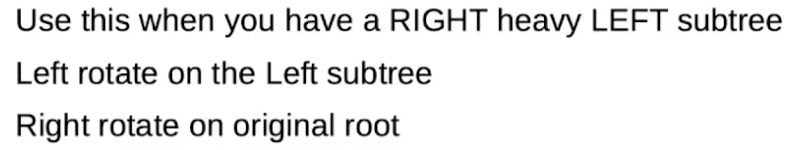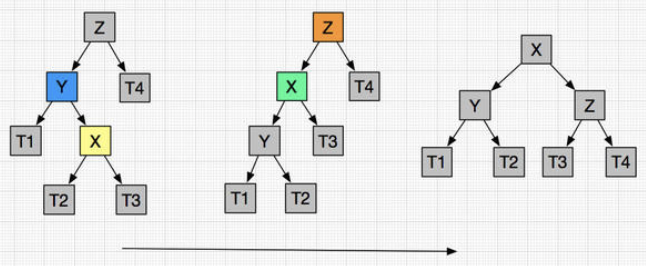### 2.3 二叉堆, 优先序列, 跳跃表和并查集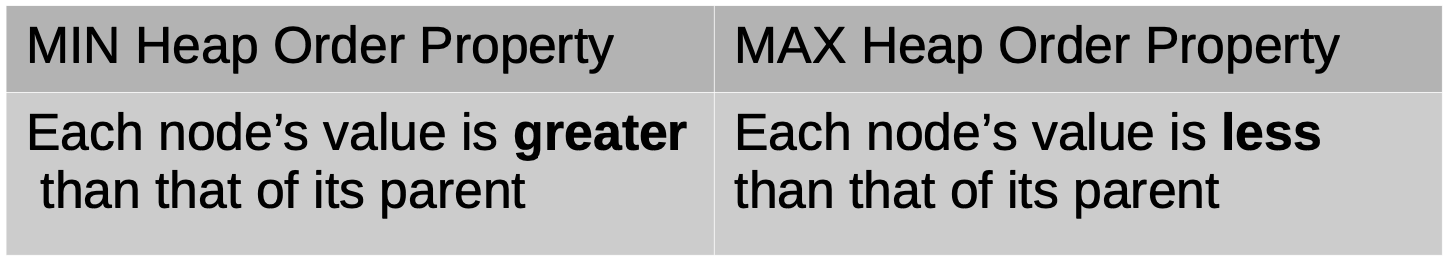1. 向堆中插入元素 (本质是新节点的上浮)

在向现存的二叉堆插入元素时, 我们需要 将元素添加到表示堆的数组的末尾, 形式上就是: 将新节点 作为倒数第二层中, 从左往右数第一个度不为 $2$ 的节点的子节点.

在将元素插入后, 我们需要对 整个堆 都重新维护它的 堆序性质:我们需要 自底向上 地依次检查子树的堆序, 直到检查指针上浮到根节点为止.

记堆包含 $n$ 个元素, 则它是一棵高为 $\log(n)$ 的二叉树, 因此插入函数的时间复杂度为 $O(\log(n))$.

2. 从堆中删除元素 (本质是最大堆的最后一个节点的下沉)

我们只能 从最大堆中移除最大值. 在删除根节点后, 堆被拆分成了两棵树. 此时我们需要取 子树的最后一个节点 充当树的根节点. 在此之后, 自顶向下 地递归维护树的 堆序性质, 不难看出删除函数的时间复杂度也为 $O(\log(n))$.

3. 创建最大堆的本质就是 自底向上 地维护某棵二叉树.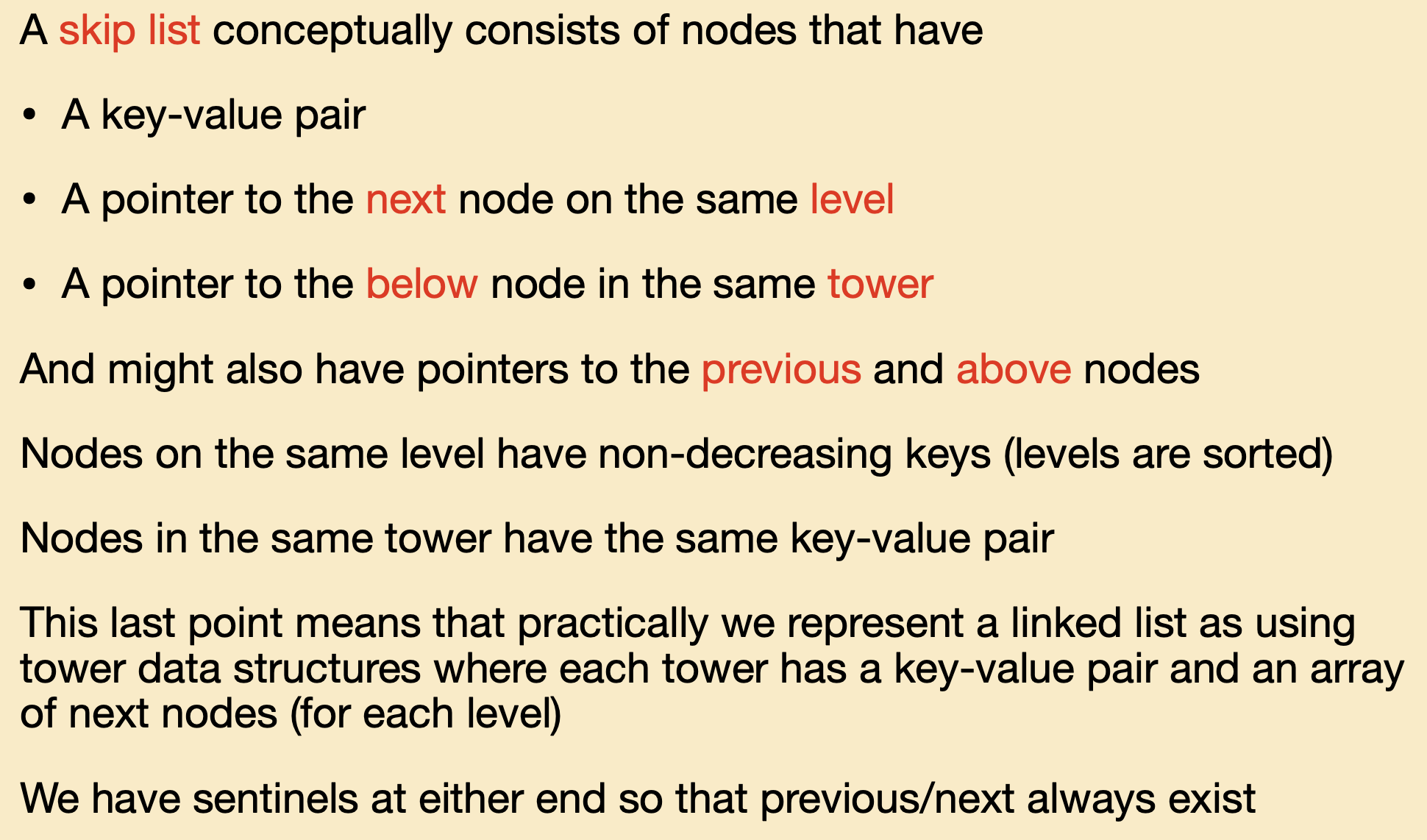1. 将新节点插入到原链表中.
2. 对这个新节点, 使用 抛硬币 (Coin Flipping) 的方式决定它将被提升为哪一级的索引:连续 “抛硬币” (模拟抛硬币), 直到抛出 $0$ 为止, 在此之前抛出了多少个 $1$ 就将这个节点提升为哪一级的索引.

1. 跳跃表的索引是在 每一次插入新数据和删除旧数据时 都被维护的.

2. 在一般情况下, 跳跃表的插入与删除的时间复杂度均为 $O(\log(n))$, 而跳跃表的空间复杂度 (所占空间) 为 $O(N)$ (实际上由于每个元素的期望高度为 $2$, 其实际占用空间应该是2N).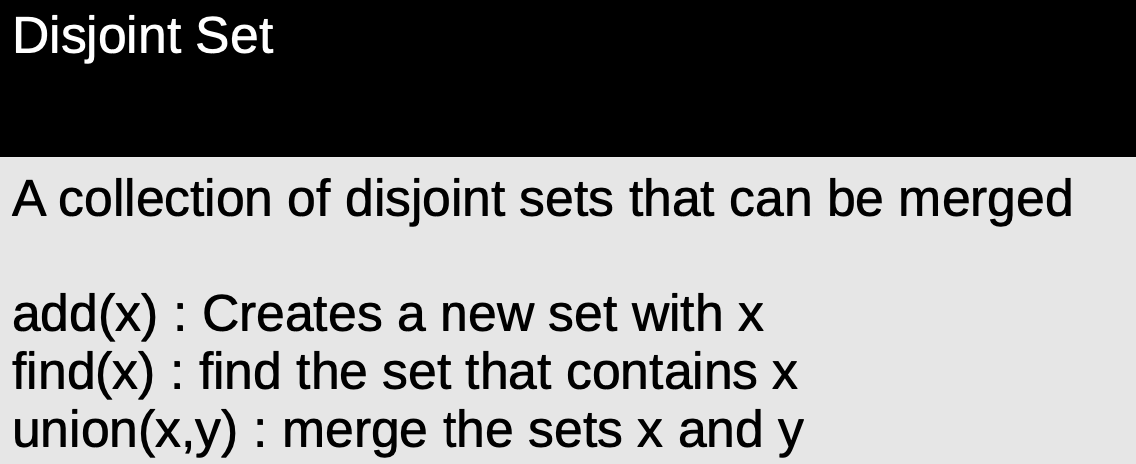1. Path Splitting:

对某并查集的某个元素执行 Path Splitting 时, 我们将无差别地将 从该元素到根节点的路径上每个节点的父节点都替换为它的祖父节点.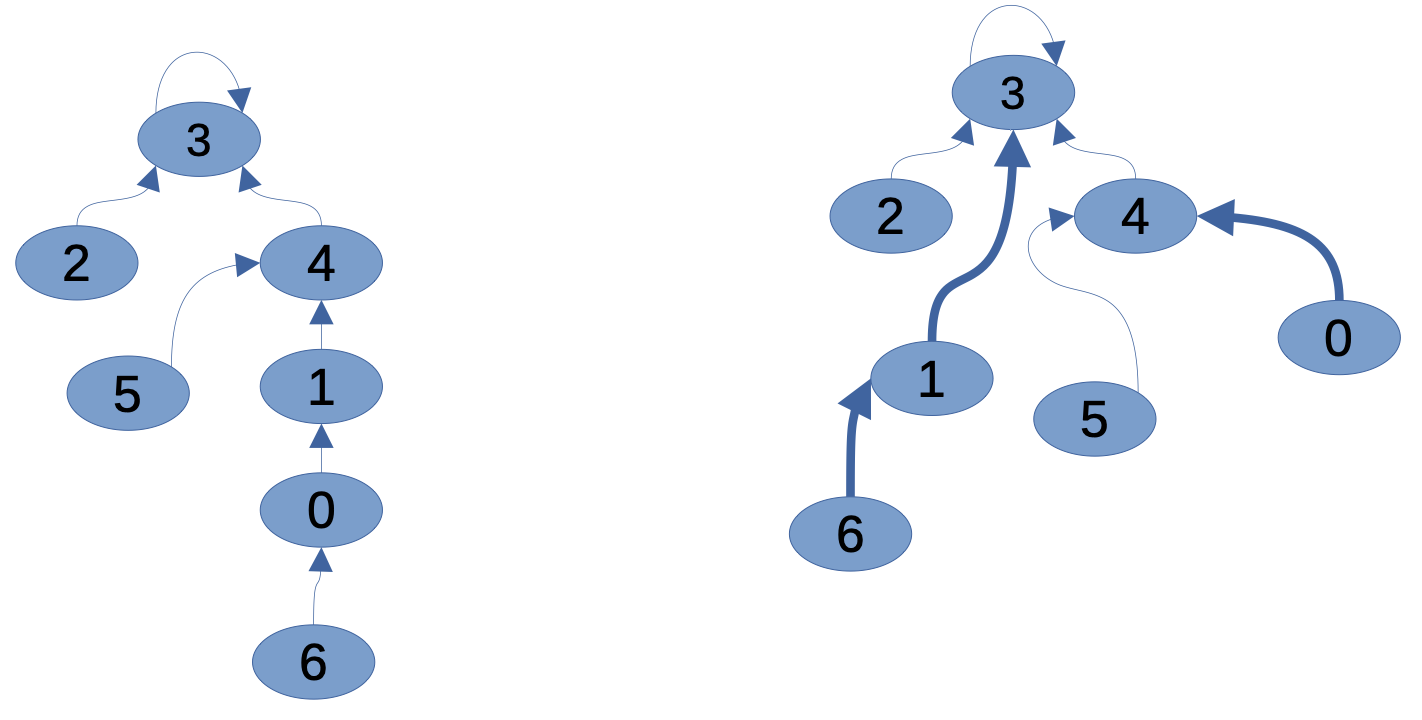2. Path Halving:

对某并查集的某个元素执行 Path Halving 时, 我们只考虑 从该元素到根节点的路径上 以该元素起算的第 $1, 3, 5, …$ 个节点 (也就是该元素, 该元素的祖父节点, 该元素祖父节点的祖父节点, …), 只将它们的父节点都替换为它们的祖父节点.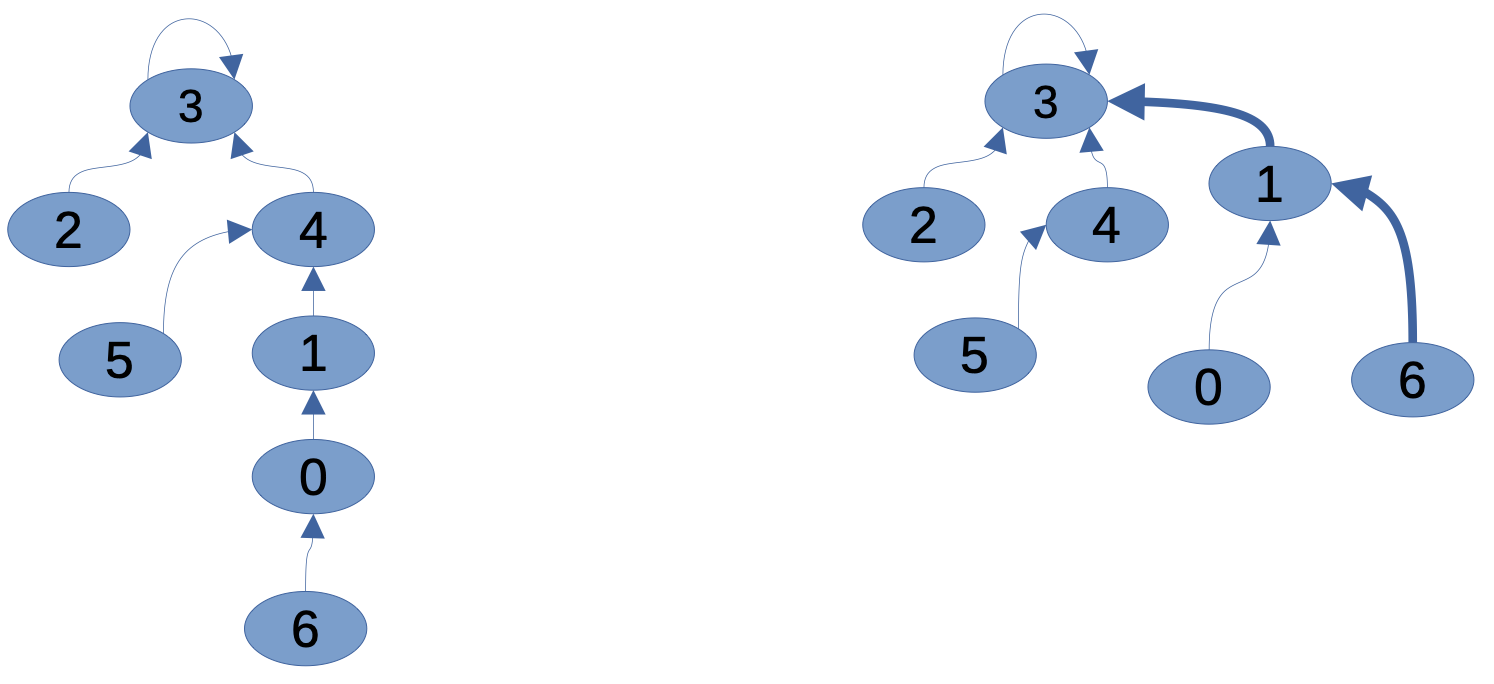3. Path Compression:

对某并查集的某个元素执行 Path Compression 时, 我们将 从该元素到根节点的路径上经过的每个节点 的父节点都替换为根节点.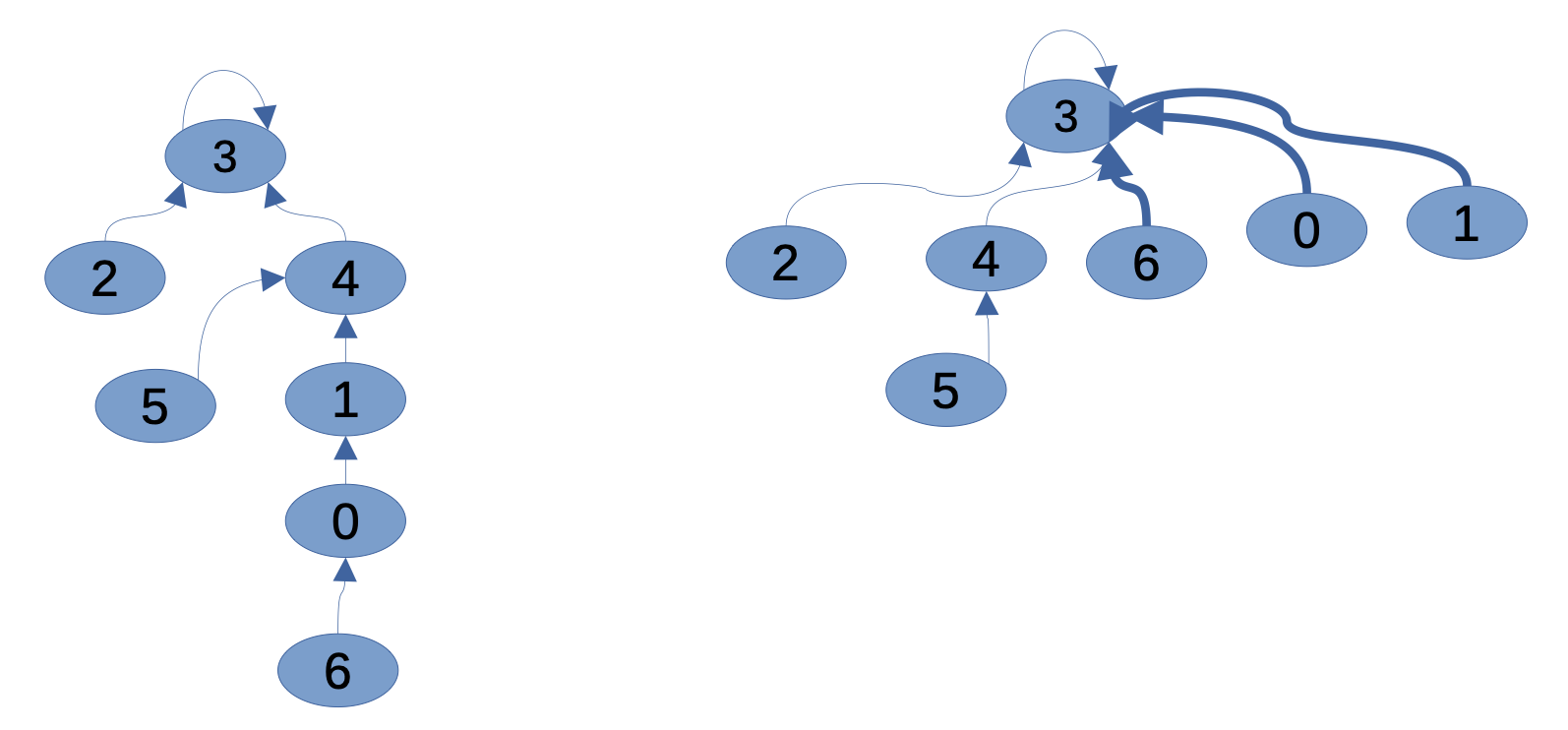## 3. 算法设计技术

1. 决策性问题: 给定输入, 算法需要给出非黑即白的答案.
2. 功能性问题: 给定输入, 算法需要给出一个解, 但解的结构按照具体问题不同而变化.
3. 搜索问题: 给定输入或条件, 算法需要从某个解空间中找出适合的答案.
4. 计数问题: 算法需要统计解空间中有多少个可行解.
5. 优化问题: 算法需要从解空间中找出相对最好的解.

### 1. 问题分解: 动态规划与分治

1. 若问题的最优解包含的子问题的解也是最优的, 也就是说该问题具有 最优子结构.
2. 在问题中, 任何状态下的决策都不会影响之前的状态.
3. 子问题之间相互重叠, 一个子问题在下一阶段的决策中也可能被用到.

### 2. 解空间搜索: 深度优先, 分支定界, 贪心搜索和启发式搜索

1. 回溯算法: 从根节点开始按照某种顺序一步步地构造可行解, 若使用某种组合构造出的解不可行则回退到上一步尝试其他方案, 并继续检查生成的新解. 重复这一过程, 最终只要原问题有解, 就必然可以得到一个可行解.
2. 分支定界算法: 在回溯算法的基础上, 维护一个 “当前最优解” 并在作出分支选择 (构造解) 时不断比较, 舍弃那些确定无法得到更优解的决策分支 (也就是剪枝 Pruning).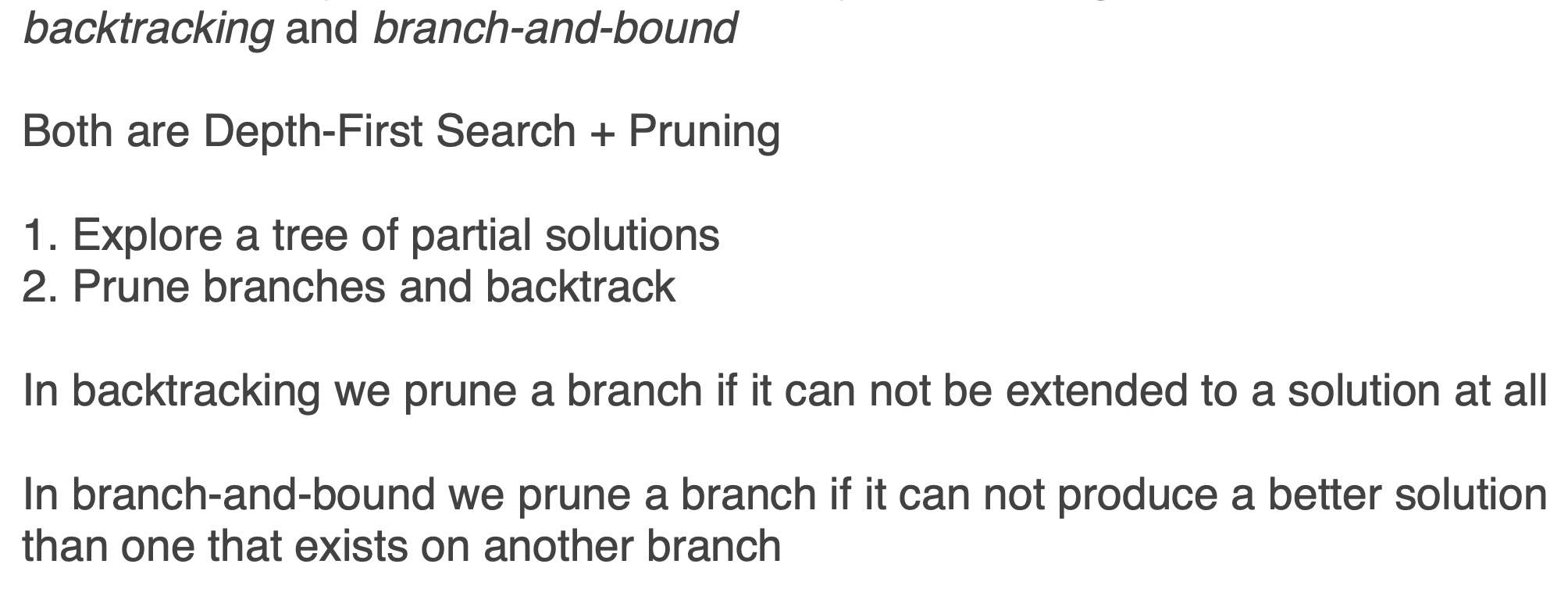1. 该问题的全局最优解可以表示为多个局部最优解的组合.
2. 或者该问题解的前部分不能被后部分所影响. (We can make a series of choices such that we never need to go back and change the choice later)

REFERENCE:

AVL树的旋转操作详解

Lecture 21: Amortized Analysis

Simple union-find techniques